The Australian Journal of Mathematical Analysis and Applications

 Home News Editors Volumes RGMIA Subscriptions Authors Contact

ISSN 1449-5910

Sorry death is imminent for file: ../public_html/searchroot/files/tex/v19n2/v19i2p4.tex
You searched for jun
Total of 449 results found in site

40: Paper Source PDF document

Paper's Title:

Some Convergence Results for  Jungck-Am Iterative Process In Hyperbolic Spaces

Author(s):

Akindele Adebayo Mebawondu and Oluwatosin Temitope Mewomo

School of Mathematics, Statistics and Computer Science,
University of KwaZulu-Natal, Durban,
South Africa.
E-mail: 216028272@stu.ukzn.ac.za, mewomoo@ukzn.ac.za

Abstract:

In this paper, we introduce a new three steps iterative process called Jungck-AM iterative process and show that the proposed iterative process can be used to approximate fixed points of Jungck-contractive type mappings and Jungck-Suzuki type mappings. In addition, we establish some strong and Δ-convergence results for the approximation of fixed points of Jungck-Suzuki type mappings in the frame work of uniformly convex hyperbolic space. Furthermore, we show that the newly proposed iterative process has a better rate of convergence compare to the Jungck-Noor, Jungck-SP, Jungck-CR and some existing iterative processes in the literature. Finally, stability, data dependency results for Jungck-AM iterative process is established and we present an analytical proof and numerical examples to validate our claim.

34: Paper Source PDF document

Paper's Title:

Ostrowski Type Inequalities for Lebesgue Integral: a Survey of Recent Results

Author(s):

Sever S. Dragomir1,2

1Mathematics, School of Engineering & Science
Victoria University, PO Box 14428
Melbourne City, MC 8001,
Australia
E-mail: sever.dragomir@vu.edu.au

2DST-NRF Centre of Excellence in the Mathematical and Statistical Sciences,
School of Computer Science & Applied Mathematics,
University of the Witwatersrand,
Private Bag 3, Johannesburg 2050,
South Africa
URL: http://rgmia.org/dragomir

Abstract:

The main aim of this survey is to present recent results concerning Ostrowski type inequalities for the Lebesgue integral of various classes of complex and real-valued functions. The survey is intended for use by both researchers in various fields of Classical and Modern Analysis and Mathematical Inequalities and their Applications, domains which have grown exponentially in the last decade, as well as by postgraduate students and scientists applying inequalities in their specific areas.

12: Paper Source PDF document

Paper's Title:

Asymptotic Behavior of Mixed Type Functional Equations

Author(s):

J. M. Rassias

Pedagogical Department, E.E., National and Capodistrian University of Athens, Section of Mathematics And Informatics, 4, Agamemnonos Str., Aghia Paraskevi, Athens 15342,Greece
jrassias@primedu.uoa.gr
URL: http://www.primedu.uoa.gr/~jrassias/

Abstract:

In 1983 Skof  was the first author to solve the Ulam problem for additive mappings on a restricted domain. In 1998 Jung  investigated the Hyers-Ulam stability of additive and quadratic mappings on restricted domains. In this paper we improve the bounds and thus the results obtained by Jung , in 1998 and by the author , in 2002. Besides we establish new theorems about the Ulam stability of mixed type functional equations on restricted domains. Finally, we apply our recent results to the asymptotic behavior of functional equations of different types.

9: Paper Source PDF document

Paper's Title:

A Fixed Point Approach to the Stability of the Equation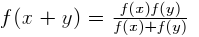Author(s):

Soon-Mo Jung

Mathematics Section, College of Science and Technology
Hong-Ik University, 339-701 Chochiwon
Republic of Korea.

smjung@hongik.ac.kr

Abstract:

We will apply a fixed point method for proving the Hyers--Ulam stability of the functional equation.

8: Paper Source PDF document

Paper's Title:

Applications of Relations and Relators in the Extensions of Stability Theorems for Homogeneous and Additive Functions

Author(s):

Árpád Száz

Institute of Mathematics, University of Debrecen,
H-4010 Debrecen, Pf. 12,
Hungary
szaz@math.klte.hu

Abstract:

By working out an appropriate technique of relations and relators and extending the ideas of the direct methods of Z. Gajda and R. Ger, we prove some generalizations of the stability theorems of D. H. Hyers, T. Aoki, Th. M. Rassias and P. Găvruţă in terms of the existence and unicity of 2-homogeneous and additive approximate selections of generalized subadditive relations of semigroups to vector relator spaces. Thus, we obtain generalizations not only of the selection theorems of Z. Gajda and R. Ger, but also those of the present author.

8: Paper Source PDF document

Paper's Title:

Ulam Stability of Reciprocal Difference and Adjoint Functional Equations

Author(s):

K. Ravi, J. M. Rassias and B. V. Senthil Kumar

Department of Mathematics,
Sacred Heart College, Tirupattur - 635601,
India

Pedagogical Department E. E.,
Section of Mathematics and Informatics,
National and Capodistrian University of Athens,
Athens, Attikis 15342,
GREECE

Department of Mathematics,
C.Abdul Hakeem College of Engineering and
Technology, Melvisharam - 632 509, India

shckavi@yahoo.co.in
jrassias@primedu.uoa.gr
bvssree@yahoo.co.in

Abstract:

In this paper, the reciprocal difference functional equation (or RDF equation) and the reciprocal adjoint functional equation (or RAF equation) are introduced. Then the pertinent Ulam stability problem for these functional equations is solved, together with the extended Ulam (or Rassias) stability problem and the generalized Ulam (or Ulam-Gavruta-Rassias) stability problem for the same equations.

7: Paper Source PDF document

Paper's Title:

Approximation of an AQCQ-Functional Equation and its Applications

Author(s):

Choonkil Park and Jung Rye Lee

Department of Mathematics,
Research Institute for Natural Sciences,
Hanyang University, Seoul 133-791,
Korea;

Department of Mathematics,
Daejin University,
Kyeonggi 487-711,
Korea

baak@hanyang.ac.kr
jrlee@daejin.ac.kr

Abstract:

This paper is a survey on the generalized Hyers-Ulam stability of an AQCQ-functional equation in several spaces. Its content is divided into the following sections:

1. Introduction and preliminaries.

2. Generalized Hyers-Ulam stability of an AQCQ-functional equation in Banach spaces: direct method.

3. Generalized Hyers-Ulam stability of an AQCQ-functional equation in Banach spaces: fixed point method.

4. Generalized Hyers-Ulam stability of an AQCQ-functional equation in random Banach spaces: direct method.

5. Generalized Hyers-Ulam stability of an AQCQ-functional equation in random Banach spaces: fixed point method.

6. Generalized Hyers-Ulam stability of an AQCQ-functional equation in non-Archi-medean Banach spaces: direct method.

7. Generalized Hyers-Ulam stability of an AQCQ-functional equation in non-Archi-medean Banach spaces: fixed point method.

6: Paper Source PDF document

Paper's Title:

New Coincidence and Fixed Point Theorems for Strictly Contractive Hybrid Maps

Author(s):

S. L. Singh and Amal M. Hashim

21, Govind Nagar, Rishikesh 249201,
Ua, India
vedicmri@sancharnet.in

Dept. of Math., College of Science,
Univ. of Basarah,
Iraq.

Abstract:

The purpose of this paper is to study the (EA)-property and noncompatible maps of a hybrid pair of single-valued and multivalued maps in fixed point considerations. Such maps have the remarkable property that they need not be continuous at their common fixed points. We use this property to obtain some coincidence and fixed point theorems for strictly contractive hybrid maps without using their continuity and completeness or compactness of the space.

6: Paper Source PDF document

Paper's Title:

Analysis of the Flow Field in Stenosed Bifurcated Arteries Through a Mathematical Model

Author(s):

S. Chakravarty and S. Sen

Department of Mathematics, Visva-Bharati University,
Santiniketan 731235,
India
santabrata2004@yahoo.co.in

Abstract:

The present study is dealt with an appropriate mathematical model of the arotic bifurcation in the presence of constrictions using which the physiological flow field is analized. The geometry of the bifurcated arterial segment having constrictions in both the parent and its daughter arterial lumen frequently occurring in the diseased arteries causing malfunction of the cardiovascular system , is formed mathematically with the introduction of appropriate curvatures at the lateral junctions and the flow divider. The flowing blood contained in the stenosed bifurcated artery is treated to be Newtonian and the flow is considered to be two dimensional. The motion of the arterial wall and its effect on local fluid mechanics is not ruled out from the present pursuit. The flow analysis applies the time-dependent, two-dimensional incompressible nonlinear Navier-Stokes equations for Newtonian fluid. The flow field can be obtained primarily following the radial coordinate transformation and using the appropriate boundary conditions and finally adopting a suitable finite difference scheme numerically. The influences of the arterial wall distensibility and the presence of stenosis on the flow field, the flow rate and the wall shear stresses are quantified in order to indicate the susceptibility to atherosclerotic lesions and thereby to validate the applicability of the present theoretical model.

6: Paper Source PDF document

Paper's Title:

Ulam Stability of Functional Equations

Author(s):

Stefan Czerwik and Krzysztof Król

Institute of Mathematics
Silesian University of Technology
Kaszubska 23, 44-100 Gliwice,
Poland

Stefan.Czerwik@polsl.pl
Krzysztof.Krol@polsl.pl

Abstract:

In this survey paper we present some of the main results on Ulam-Hyers-Rassias stability for important functional equations.

6: Paper Source PDF document

Paper's Title:

Common Fixed Point Results for Banach Operator Pairs and Applications to Best Approximation

Author(s):

Hemant Kumar Nashine

Department of Mathematics,
Disha Institute of Management and Technology,
Satya Vihar, Vidhansabha - Chandrakhuri Marg (Baloda Bazar Road), Mandir Hasaud,
Raipur - 492101(Chhattisgarh), India.

hemantnashine@rediffmail.com
nashine_09@rediffmail.com

Abstract:

The common fixed point results for Banach operator pair with generalized nonexpansive mappings in q-normed space have been obtained in the present work. As application, some more general best approximation results have also been determined without the assumption of linearity or affinity of mappings. These results unify and generalize various existing known results with the aid of more general class of noncommuting mappings.

6: Paper Source PDF document

Paper's Title:

Attempts to Define a Baum--Connes Map Via Localization of Categories for Inverse Semigroups

Author(s):

Bernhard Burgstaller

Departamento de Matematica,
CEP 88.040-900 Florianopolis-SC,
Brasil.
E-mail: bernhardburgstaller@yahoo.de
URL: http://mathematik.work/bernhardburgstaller/index.html

Abstract:

An induction functor in inverse semigroup equivariant KK-theory is considered, and together with %a restriction functors certain results similar to those known from the Mackey machinery are shown. It is also verified that for any so-called E-continuous inverse semigroup its equivariant KK-theory satisfies the universal property and is a triangulated category.

5: Paper Source PDF document

Paper's Title:

Stability of a Mixed Additive, Quadratic and Cubic Functional Equation In Quasi-Banach Spaces

Author(s):

Department of Mathematics, Faculty of Sciences,
University of Mohaghegh Ardabili, Ardabil,
Iran
a.nejati@yahoo.com

Faculty of Mathematical Sciences,
University of Tabriz, Tabriz,
Iran

Abstract:

In this paper we establish the general solution of a mixed additive, quadratic and cubic functional equation and investigate the Hyers--Ulam--Rassias stability of this equation in quasi-Banach spaces. The concept of Hyers-Ulam-Rassias stability originated from Th. M. Rassias' stability theorem that appeared in his paper: On the stability of the linear mapping in Banach spaces, Proc. Amer. Math. Soc. 72 (1978), 297--300.

5: Paper Source PDF document

Paper's Title:

Hyers-Ulam-Rassias Stability of a Generalized Jensen Functional Equation

Author(s):

A. Charifi, B. Bouikhalene, E. Elqorachi and A. Redouani

Department of
Mathematics, Faculty of Sciences,
Ibn Tofail University,
Kenitra, Morocco
charifi2000@yahoo.fr bbouikhalene@yahoo.fr

Department of
Mathematics, Faculty of Sciences,
Ibn Zohr University,
elqorachi@hotmail.com Redouani-ahmed@yahoo.fr

Abstract:

In this paper we obtain the Hyers-Ulam-Rassias stability for the generalized Jensen's functional equation in abelian group (G,+). Furthermore we discuss the case where G is amenable and we give a note on the Hyers-Ulam-stability of the K-spherical (n × n)-matrix functional equation.

5: Paper Source PDF document

Paper's Title:

A Generalization of a Trace Inequality for Positive Definite Matrices

Author(s):

E. V. Belmega, M. Jungers, and S. Lasaulce

Université Paris-Sud Xi, SUPELEC,
Laboratoire Des Signaux Et Systčmes,
Gif-Sur-Yvette,
France.

belmega@lss.supelec.fr
http://veronica.belmega.lss.supelec.fr

CNRS, ENSEM, CRAN, Vandoeuvre,
France.

marc.jungers@cran.uhp-nancy.fr
http://perso.ensem.inpl-nancy.fr/Marc.Jungers/

CNRS, SUPELEC, Laboratoire des Signaux et Systčmes,
Gif-Sur-Yvette,
France.

lasaulce@lss.supelec.fr
http://samson.lasaulce.lss.supelec.fr

Abstract:

In this note, we provide a generalization of the trace inequality derived in [Belmega].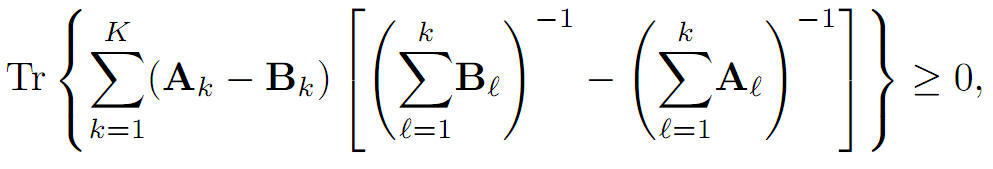More precisely, we prove that for arbitrary K ≥ 1 where Tr(∙) denotes the matrix trace operator, A1, B1 are any positive definite matrices and Ak, Bk for all k∈{2,...,k}, are any positive semidefinite matrices.

5: Paper Source PDF document

Paper's Title:

On an extension of Edwards's double integral with applications

Author(s):

I. Kim, S. Jun, Y. Vyas and A. K. Rathie

Department of Mathematics Education,
Wonkwang University,
Iksan, 570-749,
Republic of Korea.

General Education Institute,
Konkuk University,
Chungju 380-701,
Republic of Korea.

Department of Mathematics, School of Engineering,
Bhatewar, Udaipur, 313601, Rajasthan State,
India.

Department of Mathematics,
Vedant College of Engineering and Technology,
(Rajasthan Technical University),
Bundi-323021, Rajasthan,
India.

E-mail: iki@wku.ac.kr sjun@kku.ac.kr
yashoverdhan.vyas@spsu.ac.in arjunkumarrathie@gmail.com

Abstract:

The aim of this note is to provide an extension of the well known and useful Edwards's double integral. As an application, new class of twelve double integrals involving hypergeometric function have been evaluated in terms of gamma function. The results are established with the help of classical summation theorems for the series 3F2 due to Watson, Dixon and Whipple. Several new and interesting integrals have also been obtained from our main findings.

5: Paper Source PDF document

Paper's Title:

Existence of Solution of Differential and Riemann-Liouville Equation Via Fixed Point Approach in Complex Valued b-Metric Spaces

Author(s):

K. Afassinou, A. A. Mebawondu, H. A. Abass and O. K. Narain

Department of Science Access,
South Africa.
E-mail: komia@aims.ac.za

DST-NRF Centre of Excellence in Mathematical and Statistical Sciences (CoE-MaSS),
Johannesburg,
South Africa.
E-mail: dele@aims.ac.za

DST-NRF Centre of Excellence in Mathematical and Statistical Sciences (CoE-MaSS),
Johannesburg,
South Africa.
E-mail: hammedabass548@gmail.com

School of Mathematics, Statistics and Computer Science,
University of KwaZulu-Natal, Durban,
South Africa.
E-mail: naraino@ukzn.ac.za

Abstract:

In this paper, we establish some fixed point and common fixed point results for a new type of generalized contractive mapping using the notion of C-class function in the framework of complex valued b-metric spaces. As an application, we establish the existence and uniqueness of a solution for Riemann-Liouville integral and ordinary differential equation in the framework of a complete complex valued b-metric spaces. The obtained results generalize and improve some fixed point results in the literature.

4: Paper Source PDF document

Paper's Title:

Pseudomonotonicity and Quasimonotonicity by Translations versus Monotonicity in Hilbert Spaces

Author(s):

George Isac and Dumitru Motreanu

Department of Mathematics, Royal Military College of Canada, P.O. Box 17000 Stn Forces

Département de Mathématiques, Université de Perpignan, 66860 Perpignan, France.

Abstract:

Let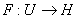be a Gâteaux differentiable mapping on an open convex subset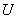of a Hilbert space. If there exists a straight line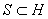such that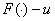is pseudomonotone for any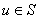then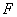is monotone. Related results using a regularity condition are given.

4: Paper Source PDF document

Paper's Title:

Komatu Integral Transforms of Analytic Functions Subordinate to Convex Functions

Author(s):

T. N. Shanmugam and C. Ramachandran

Department of Mathematics, College of Engineering,
India
shan@annauniv.edu

Department of Mathematics, College of Engineering,
India
crjsp2004@yahoo.com

Abstract:

In this paper, we consider the class A of the functions f(z) of the form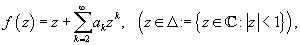which are analytic in an open disk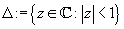and study certain subclass of the class A, for which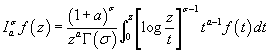has some property. Certain inclusion and the closure properties like convolution with convex univalent function etc. are studied.

4: Paper Source PDF document

Paper's Title:

On the Generalized Stability and Asymptotic Behavior of Quadratic Mappings

Author(s):

Hark-Mahn Kim, Sang-Baek Lee and Eunyoung Son

Department of Mathematics
Chungnam National University
Daejeon, 305-764,
Republic of Korea

hmkim@cnu.ac.kr

Abstract:

We extend the stability of quadratic mappings to the stability of general quadratic mappings with several variables, and then obtain an improved asymptotic property of quadratic mappings on restricted domains.

4: Paper Source PDF document

Paper's Title:

Stability Problems for Generalized Additive Mappings and Euler-Lagrange Type Mappings

Author(s):

M. Todoroki, K. Kumahara, T. Miura and S.-E. Takahasi

The Open University of Japan,
Chiba, 261-8586,
Japan
tomamiyu3232@sky.sannet.ne.jp
kumahara@ouj.ac.jp

Yamagata University,
Yonezawa 992-8510,
Japan
miura@yz.yamagata-u.ac.jp

Toho University, Yamagata University,
Chiba, 273-0866,
Japan
sin_ei1@yahoo.co.jp

Abstract:

We introduce a generalized additivity of a mapping between Banach spaces and establish the Ulam type stability problem for a generalized additive mapping. The obtained results are somewhat different from the Ulam type stability result of Euler-Lagrange type mappings obtained by H. -M. Kim, K. -W. Jun and J. M. Rassias.

4: Paper Source PDF document

Paper's Title:

A Geometric Generalization of Busemann-Petty Problem

Author(s):

Liu Rong and Yuan Jun

Shanghai Zhangjiang Group Junior Middle School,
China

Abstract:

The norm defined by Busemann's inequality establishes a class of star body - intersection body. This class of star body plays a key role in the solution of Busemann-Petty problem. In 2003, Giannapoulos  defined a norm for a new class of half-section. Based on this norm, we give a geometric generalization of Busemann-Petty problem, and get its answer as a result

4: Paper Source PDF document

Paper's Title:

Introducing the Dorfmanian: A Powerful Tool for the Calculus Of Variations

Author(s):

Olivier de La Grandville

Department of Management Science and Engineering,
Stanford University,
475 Via Ortega, Stanford, CA 94305,
U. S. A.

E-mail: odelagrandville@gmail.com

Abstract:

We show how a modified Hamiltonian proposed by Robert Dorfman  to give intuitive sense to the Pontryagin maximum principle can be extended to easily obtain all high-order equations of the calculus of variations. This new concept is particularly efficient to determine the differential equations leading to the extremals of functionals defined by n-uple integrals, while a traditional approach would require -- in some cases repeatedly -- an extension of Green's theorem to n-space.
Our paper is dedicated to the memory of Robert Dorfman (1916 - 2002).

4: Paper Source PDF document

Paper's Title:

Characterization of Caristi Type Mapping Through its Absolute Derivative

Author(s):

M. Muslikh1, A. Kilicman2,3, S. H. Sapar4 and N. Bacho5

1Department of Mathematics,
University of Brawijaya,
Malang 65143, East Java,
Indonesia.
E-mail: mslk@ub.ac.id

2Department of Mathematics,
Universiti Putra Malaysia,
43400 UPM, Serdang, Selangor,
Malaysia
E-mail: akilic@upm.edu.my

3Department of Electrical and Electronic Engineering,
Istanbul Gelisim University,
Avcilar, Istanbul,
Turkey

4Department of Mathematics,
Universiti Putra Malaysia,
43400 UPM, Serdang, Selangor,
Malaysia
E-mail: sitihas@upm.edu.my

5Department of Mathematics,
Universiti Putra Malaysia,
43400 UPM, Serdang, Selangor,
Malaysia
E-mail: norfifah@upm.edu.my

Abstract:

The purpose of this article to characterize the Caristi type mapping by the absolute derivative. The equivalences of the Caristi mapping with contraction mapping is discussed too. In addition, it was shown that the contraction mapping can be tested through its absolute derivative.

4: Paper Source PDF document

Paper's Title:

Sharp Inequalities Between Hölder and Stolarsky Means of Two Positive Numbers

Author(s):

M. Bustos Gonzalez and A. I. Stan

The University of Iowa,
Department of Mathematics,
14 MacLean Hall,
Iowa City, Iowa,
USA.
E-mail: margarita-bustosgonzalez@uiowa.edu

The Ohio State University at Marion,
Department of Mathematics,
1465 Mount Vernon Avenue,
Marion, Ohio,
USA.
E-mail: stan.7@osu.edu

Abstract:

Given any index of the Stolarsky means, we find the greatest and least indexes of the H\"older means, such that for any two positive numbers, the Stolarsky mean with the given index is bounded from below and above by the Hölder means with those indexes, of the two positive numbers. Finally, we present a geometric application of this inequality involving the Fermat-Torricelli point of a triangle.

4: Paper Source PDF document

Paper's Title:

Pointwise Convergence of Fourier-type Series with Exponential Weights

Author(s):

Hee Sun Jung and Ryozi Sakai

Department of Mathematics Education, Sungkyunkwan University,
Seoul 110-745,
Republic of Korea.
E-mail: hsun90@skku.edu

Department of Mathematics,
Meijo University, Nagoya 468-8502,
Japan.
E-mail: ryozi@hm.aitai.ne.jp

Abstract:

Let R = ( - ∞,∞), and let Q∈C1(R):R→[0,∞) be an even function. We consider the exponential weights w(x)=e-Q(x), xR. In this paper we obtain a pointwise convergence theorem for the Fourier-type series with respect to the orthonormal polynomials {pn(w2;x)}.

3: Paper Source PDF document

Paper's Title:

Fixed Point Theorems for a Finite Family of Asymptotically Nonexpansive Mappings

Author(s):

E. Prempeh

Department of Mathematics,
Kwame Nkrumah University of Science and Technology,
Kumasi, Ghana
edward_prempeh2000@yahoo.com

Abstract:

Let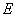be a real reflexive Banach space with a uniformly Gâteaux differentiable norm,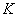be a nonempty bounded closed convex subset of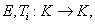i=1,2,...,r be a finite family of asymptotically nonexpansive mappings such that for each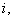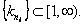Let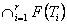be a nonempty set of common fixed points of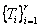and define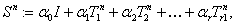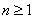. Let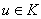be fixed and let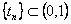be such that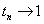as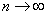. We can prove that the sequence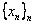satisfying the relation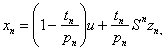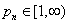associated with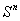, converges strongly to a fixed point of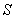providedpossesses uniform normal structure. Furthermore we prove that the iterative process: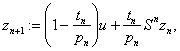, converges strongly to a fixed point of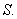3: Paper Source PDF document

Paper's Title:

Solution of the Hyers-Ulam Stability Problem for Quadratic Type Functional Equations in Several Variables

Author(s):

John Michael Rassias

Pedagogical Department, E.E., National and Capodistrian University of Athens,
Section of Mathematics and Informatics,
Athens 15342,
Greece jrassias@primedu.uoa.gr
URL: http://www.primedu.uoa.gr/~jrassias/

Abstract:

In 1940 (and 1968) S. M. Ulam proposed the well-known Ulam stability problem. In 1941 D. H. Hyers solved the Hyers-Ulam problem for linear mappings. In 1951 D. G. Bourgin has been the second author treating the Ulam problem for additive mappings. In 1978 according to P. M. Gruber this kind of stability problems is of particular interest in probability theory and in the case of functional equations of different types. In 1982-2004 we established the Hyers-Ulam stability for the Ulam problem for different mappings. In this article we solve the Hyers-Ulam problem for quadratic type functional equations in several variables. These stability results can be applied in stochastic analysis, financial and actuarial mathematics, as well as in psychology and sociology.

3: Paper Source PDF document

Paper's Title:

A general common fixed point theorem for reciprocally continuous mappings satisfying an implicit relation

Author(s):

A. Djoudi and A. Aliouche

Faculty of Science, University of Annaba,
P.O. Box 23000, Annaba,
Algeria.

Department of Mathematics, University of Larbi Ben M'Hidi,
Oum-El-Bouaghi 04000,
Algeria.
abdmath@hotmail.com

Abstract:

A general common fixed point theorem for compatible mappings satisfying an implicit relation is obtained by replacing the continuity of one mapping by the reciprocal continuity of two mappings.

3: Paper Source PDF document

Paper's Title:

A Stability of the G-type Functional Equation

Author(s):

Gwang Hui Kim

Department of Mathematics, Kangnam University
Suwon 449-702, Korea.
ghkim@kangnam.ac.kr

Abstract:

We will investigate the stability in the sense of Găvruţă for the G-type functional equation f(φ(x))=Γ(x)f(x)+ψ(x) and the stability in the sense of Ger for the functional equation of the form f(φ(x))=Γ(x)f(x). As a consequence, we obtain a stability results for G-function equation.

3: Paper Source PDF document

Paper's Title:

Generalizations of Hermite-Hadamard's Inequalities for Log-Convex Functions

Author(s):

Ai-Jun Li

School of Mathematics and Informatics, Henan Polytechnic University,
Jiaozuo City, Henan Province,
454010, China.
liaijun72@163.com

Abstract:

In this article, Hermite-Hadamard's inequalities are extended in terms of the weighted power mean and log-convex function. Several refinements, generalizations and related inequalities are obtained.

3: Paper Source PDF document

Paper's Title:

On the Generalized Inverse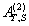over Integral Domains

Author(s):

Yaoming Yu and Guorong Wang

College of Education, Shanghai Normal University
Shanghai 200234
People's Republic of China.
yuyaoming@online.sh.cn
grwang@shnu.edu.cn

Abstract:

In this paper, we study further the generalized inverseof a matrix A over an integral domain. We give firstly some necessary and sufficient conditions for the existence of the generalized inverse, an explicit expression for the elements of the generalized inverseand an explicit expression for the generalized inverse, which reduces to the {1} inverse. Secondly, we verify that the group inverse, the Drazin inverse, the Moore-Penrose inverse and the weighted Moore-Penrose inverse are identical with the generalized inverse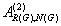for an appropriate matrix G, respectively, and then we unify the conditions for the existence and the expression for the elements of the weighted Moore-Penrose inverse, the Moore-Penrose inverse, the Drazin inverse and the group inverse over an integral domain. Thirdly, as a simple application, we give the relation between some rank equation and the existence of the generalized inverse, and a method to compute the generalized inverse. Finally, we give an example of evaluating the elements ofwithout calculating.

3: Paper Source PDF document

Paper's Title:

A Subclass of Meromorphically Multivalent Functions with Applications to Generalized Hypergeometric Functions

Author(s):

M. K. Aouf

Mathematics Department, Faculty of Science,
Mansoura University 35516,
Egypt
mkaouf127@yahoo.com

Abstract:

In this paper a new subclass of meromorphically multivalent functions, which is defined by means of a Hadamard product (or convolution) involving some suitably normalized meromorphically p-valent functions. The main object of the present paper is to investigate the various important properties and characteristics of this subclass of meromorphically multivalent functions. We also derive many interesting results for the Hadamard products of functions belonging to this subclass. Also we consider several applications of our main results to generalized hypergeomtric functions.

3: Paper Source PDF document

Paper's Title:

Some Identities for Ramanujan - Göllnitz - Gordon Continued fraction

Author(s):

M. S. Mahadeva Naika, B. N. Dharmendra and S. Chandan Kumar

Department of Mathematics,
Bangalore University,
Central College Campus,
Bangalore-560 001,
INDIA

msmnaika@rediffmail.com

chandan.s17@gmail.com

Department of Mathematics,
Maharani's Science College for Women,
J. L. B. Road, Mysore-570 001,
INDIA

dharmamath@rediffmail.com

Abstract:

In this paper, we obtain certain P--Q eta--function identities, using which we establish identities providing modular relations between Ramanujan-Göllnitz-Gordon continued fraction H(q) and H(q^n) for n= 2, 3, 4, 5, 7, 8, 9, 11, 13, 15, 17, 19, 23, 25, 29 and 55.

3: Paper Source PDF document

Paper's Title:

On a New Class of Eulerian's Type Integrals Involving Generalized Hypergeometric Functions

Author(s):

Sungtae Jun, Insuk Kim and Arjun K. Rathie

General Education Institute,
Konkuk University, Chungju 380-701,
Republic of Korea.

Department of Mathematics Education,
Wonkwang University, Iksan, 570-749,
Republic of Korea.

Department of Mathematics,
Vedant College of Engineering and Technology (Rajasthan Technical University),
Bundi-323021, Rajasthan,
India.

E-mail: sjun@kku.ac.kr, iki@wku.ac.kr, arjunkumarrathie@gmail.com

Abstract:

Very recently Masjed-Jamei and Koepf established interesting and useful generalizations of various classical summation theorems for the 2F1, 3F2, 4F3, 5F4 and 6F5 generalized hypergeometric series. The main aim of this paper is to establish eleven Eulerian's type integrals involving generalized hypergeometric functions by employing these theorems. Several special cases have also been given.

3: Paper Source PDF document

Paper's Title:

Formulation of Approximate Mathematical Model for Incoming Water to Some Dams on Tigris and Euphrates Rivers Using Spline Function

Author(s):

Nadia M. J. Ibrahem, Heba A. Abd Al-Razak, and Muna M. Mustafa

Mathematics Department,
College of Sciences for Women,
Iraq.

Abstract:

In this paper, we formulate three mathematical models using spline functions, such as linear, quadratic and cubic functions to approximate the mathematical model for incoming water to some dams. We will implement this model on dams of both rivers; dams on the Tigris are Mosul and Amara while dams on the Euphrates are Hadetha and Al-Hindya.

3: Paper Source PDF document

Paper's Title:

On Euler's First Transformation Formula for k-hypergeometric Function

Author(s):

Sungtae Jun and Insuk Kim

General Education Institute,
Konkuk University, Chungju 380-701,
Republic of Korea.
E-mail: sjun@kku.ac.kr

Department of Mathematics Education,
Wonkwang University, Iksan, 570-749,
Republic of Korea.
E-mail: iki@wku.ac.kr

Abstract:

Mubeen et al. obtained Kummer's first transformation for the k-hypergeometric function. The aim of this note is to provide the Euler-type first transformation for the k-hypergeometric function. As a limiting case, we recover the results of Mubeen et al. In addition to this, an alternate and easy derivation of Kummer's first transformation for the k-hypergeometric function is also given.

3: Paper Source PDF document

Paper's Title:

A Self Adaptive Method for Solving Split Bilevel Variational Inequalities Problem in Hilbert Spaces

Author(s):

Francis Akutsah1, Ojen Kumar Narain2, Funmilayo Abibat Kasali3 Olawale Kazeem Oyewole4 and Akindele Adebayo Mebawondu5

1School of Mathematics,
Statistics and Computer Science,
University of KwaZulu-Natal, Durban,
South Africa.
E-mail: 216040405@stu.ukzn.ac.za, akutsah@gmail.com

2School of Mathematics,
Statistics and Computer Science,
University of KwaZulu-Natal, Durban,
South Africa.
E-mail: naraino@ukzn.ac.za

3Mountain Top University,
Prayer City, Ogun State,
Nigeria.
E-mail: fkasali@mtu.edu.ng

4Technion-Israel Institute of Technology.
E-mail: 217079141@stu.ukzn.ac.za, oyewoleolawalekazeem@gmail.co

5School of Mathematics,
Statistics and Computer Science,
University of KwaZulu-Natal, Durban,
South Africa.
DST-NRF Centre of Excellence in Mathematical and Statistical Sciences (CoE-MaSS),
Johannesburg,
South Africa.
Mountain Top University,
Prayer City, Ogun State,
Nigeria.
E-mail: dele@aims.ac.za

Abstract:

In this work, we study the split bilevel variational inequality problem in two real Hilbert spaces. We propose a new modified inertial projection and contraction method for solving the aforementioned problem when one of the operators is pseudomonotone and Lipschitz continuous while the other operator is α-strongly monotone. The use of the weakly sequential continuity condition on the Pseudomonotone operator is removed in this work. A Strong convergence theorem of the proposed method is proved under some mild conditions. In addition, some numerical experiments are presented to show the efficiency and implementation of our method in comparison with other methods in the literature in the framework of infinite dimensional Hilbert spaces. The results obtained in this paper extend, generalize and improve several.

2: Paper Source PDF document

Paper's Title:

Integrability of Sine and Cosine Series Having Coefficients of a New Class

Author(s):

L. Leindler

Bolyai Institute, University of Szeged, Aradi Vértanúk Tere 1, H-6720 Szeged, Hungary
leindler@math.u-szeged.hu

Abstract:

Some integrability theorems or only their sufficient part are generalized such that the coefficients of the sine and cosine series belong to a new class of sequences being wider than the class of sequences of rest bounded variation, which itself is a generalization of the monotone decreasing sequences, but a subclass of the almost monotone decreasing sequences. It is also verified that the new class of sequences and the class of almost monotone decreasing sequences are not comparable.

2: Paper Source PDF document

Paper's Title:

Viability Theory And Differential Lanchester Type Models For Combat.
Differential Systems.

Author(s):

G. Isac and A. Gosselin

Department Of Mathematics, Royal Military College Of Canada,
P.O. Box 17000, S
tn Forces, Kingston, Ontario, Canada K7k 7b4

URL
:

URL
:

Abstract:

In 1914, F.W. Lanchester proposed several mathematical models based on differential equations to describe combat situations . Since then, his work has been extensively modified to represent a variety of competitions including entire wars. Differential Lanchester type models have been studied from many angles by many authors in hundreds of papers and reports. Lanchester type models are used in the planning of optimal strategies, supply and tactics. In this paper, we will show how these models can be studied from a viability theory stand point. We will introduce the notion of winning cone and show that it is a viable cone for these models. In the last part of our paper we will use the viability theory of differential equations to study Lanchester type models from the optimal theory point of view.

2: Paper Source PDF document

Paper's Title:

On the Ulam Stability for Euler-Lagrange Type Quadratic Functional Equations

Author(s):

Matina John Rassias and John Michael Rassias

Statistics and Modelling Science,
University of Strathclyde,
Livingstone Tower,
26 Richmond Str,
Glasgow, Uk, G1 1xh

Pedagogical Department, E. E., National and Capodistrian University of Athens,
Section of Mathematics and Informatics,
Athens 15342, Greece

Abstract:

In 1940 (and 1968) S. M. Ulam proposed the well-known Ulam stability problem. In 1941 D.H. Hyers solved the Hyers-Ulam problem for linear mappings. In 1951 D. G. Bourgin has been the second author treating the Ulam problem for additive mappings. In 1978 according to P.M. Gruber this kind of stability problems is of particular interest in probability theory and in the case of functional equations of different types. In 1982-2004 we established the Hyers-Ulam stability for the Ulam problem for different mappings. In 1992-2000 J.M. Rassias investigated the Ulam stability for Euler-Lagrange mappings. In this article we solve the Ulam problem for Euler-Lagrange type quadratic functional equations. These stability results can be applied in mathematical statistics, stochastic analysis, algebra, geometry, as well as in psychology and sociology.

2: Paper Source PDF document

Paper's Title:

Classes of Meromorphic p-valent Parabolic Starlike Functions with Positive Coefficients

Author(s):

S. Sivaprasad Kumar, V. Ravichandran, and G. Murugusundaramoorthy

Department of Applied Mathematics
Delhi College of Engineering,
Delhi 110042, India
sivpk71@yahoo.com

School of Mathematical Sciences
Universiti Sains Malaysia
11800 USM Penang
Malaysia
vravi@cs.usm.my
URL: http://cs.usm.my/~vravi

Department of Mathematics
Vellore Institute of Technology (Deemed University)
Vellore 632 014, India
gmsmoorthy@yahoo.com

Abstract:

In the present paper, we consider two general subclasses of meromorphic p-valent starlike functions with positive coefficients and obtain a necessary and sufficient condition for functions to be in these classes. Also we obtain certain other related results as a consequences of our main results.

2: Paper Source PDF document

Paper's Title:

Distortion Theorems for Certain Analytic Functions Involving the Coefficient Inequalities

Author(s):

Shigeyoshi Owa and Junichi Nishiwaki

Department of Mathematics, Kinki University,
Higashi-Osaka, Osaka 577-8502,
Japan

Abstract:

By virtue of the coefficient inequalities for certain analytic functions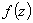in the open unit disk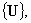two subclasses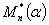and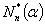are introduced. The object of the present paper is to discuss the distortion thorems of functionsbelonging to the classesinvolving the coefficient inequalities.

2: Paper Source PDF document

Paper's Title:

Coincidences and Fixed Points of Hybrid Maps in Symmetric Spaces

Author(s):

S. L. Singh and Bhagwati Prasad

Vedic MRI, 21 Govind Nagar,
Rishikesh 249201
India
vedicmri@gmail.com

Department of Mathematics, Gurukula Kangri University,
Hardwar 249404,
India

Abstract:

The purpose of this paper is to obtain a new coincidence theorem for a single-valued and two multivalued operators in symmetric spaces. We derive fixed point theorems and discuss some special cases and applications.

2: Paper Source PDF document

Paper's Title:

Product Formulas Involving Gauss Hypergeometric Functions

Author(s):

Edward Neuman

Department of Mathematics, Mailcode 4408,
Southern Illinois University,
1245 Lincoln Drive,
Carbondale, IL 62901,
USA.
edneuman@math.siu.edu
URL: http://www.math.siu.edu/neuman/personal.html

Abstract:

New formulas for a product of two Gauss hypergeometric functions are derived. Applications to special functions, with emphasis on Jacobi polynomials, Jacobi functions, and Bessel functions of the first kind, are included. Most of the results are obtained with the aid of the double Dirichlet average of a univariate function.

2: Paper Source PDF document

Paper's Title:

Isoperimetric Inequalities for Dual Harmonic Quermassintegrals

Author(s):

Yuan Jun, Zao Lingzhi and Duan Xibo

School of Mathematics and Computer Science,
Nanjing Normal University, Nanjing, 210097,
China.
yuanjun_math@126.com

Department of Mathematics, Nanjing Xiaozhuang University,
Nanjing, 211171,
China.
lzhzhao@163.com

Department of Mathematics, Shandong Water Polytechnic,
Shandong, 276826,
China
dxb1111@sohu.com

Abstract:

In this paper, some isoperimetric inequalities for the dual harmonic quermassintegrals are established.

2: Paper Source PDF document

Paper's Title:

On Stan Ulam and his Mathematics

Author(s):

Krzysztof Ciesielski and Themistocles M. Rassias

Mathematics Institute, Jagiellonian University,
Ł
jasiewicza 6, 30-348 Kraków,
Poland
Department of Mathematics. National Technical University of Athens,
Zografou Campus, 15780 Athens,
Greece

Krzysztof.Ciesielski@im.uj.edu.pl
trassias@math.ntua.gr

Abstract:

In this note we give a glimpse of the curriculum vitae of Stan Ulam, his personality and some of the mathematics he was involved in.

2: Paper Source PDF document

Paper's Title:

A One-Line Derivation of the Euler and Ostrogradski Equations

Author(s):

Olivier de La Grandville

Stanford University,
Department of Management Science and Engineering,
Stanford, CA 94305,
U. S. A

Abstract:

At the very heart of major results of classical physics, the Euler and Ostrogradski equations have apparently no intuitive interpretation. In this paper we show that this is not so. Relying on Euler's initial geometric approach, we show that they can be obtained through a direct reasoning that does not imply any calculation. The intuitive approach we suggest offers two benefits: it gives immediate significance to these fundamental second-order non-linear differential equations; and second, it allows to obtain a property of the calculus of variations that does not seem to have been uncovered until now: the Euler and Ostrogradski equations can be derived not necessarily by giving a variation to the optimal function -- as is always done; one could equally well start by giving a variation to their derivative(s).

2: Paper Source PDF document

Paper's Title:

On Convergence Theorems of an Implicit Iterative Process with Errors for a Finite Family of Asymptotically quasi I-nonexpansive Mappings

Author(s):

Farrukh Mukhamedov and Mansoor Saburov

Department of Computational & Theoretical Sciences,
Faculty of Sciences, International Islamic University Malaysia,
P.O. Box, 141, 25710, Kuantan,
Malaysia

farrukh_m@iiu.edu.my

msaburov@gmail.com

http://www.iium.edu.my

Abstract:

In this paper we prove the weak and strong convergence of the implicit iterative process with errors to a common fixed point of a finite family {Tj}Ni=1 of asymptotically quasi Ij-nonexpansive mappings as well as a family of {Ij}Nj=1 of asymptotically quasi nonexpansive mappings in the framework of Banach spaces. The obtained results improve and generalize the corresponding results in the existing literature.

2: Paper Source PDF document

Paper's Title:

Certain Compact Generalizations of Well-Known Polynomial Inequalities

Author(s):

N. A. Rather and Suhail Gulzar

Department of Mathematics,
University of Kashmir, Hazratbal Srinagar-190006,
India.

Abstract:

In this paper, certain sharp compact generalizations of well-known Bernstien-type inequalities for polynomials, from which a variety of interesting results follow as special cases, are obtained.

2: Paper Source PDF document

Paper's Title:

Uniform Continuity and k-Convexity

Author(s):

Mathematics Department, Faculty of Science, J
erash University, Jerash
Jordan.

Abstract:

A closed arcwise-connected subset A of Rn is called k-convex if for each positive number a and for all elements x and y in A there is a positive number b such that if the norm of x-y is less than or equal to b then the length of the shortest curve l(x,y) in A is less than k times the norm of x-y plus a. We show that a union of two non disjoint closed finite convex subsets need not be k-convex. Let f(x) be a uniformly continuous functions on a finite number of closed subsets A_{1},...,A_{n} of R^{n} such that the union of A_{j},...,A_{n},j=1,...,n-1 is k-convex. We show that f is uniformly continuous on the union of the sets A_{i},i=1,...,n. We give counter examples if this condition is not satisfied. As a corollary we show that if f(x) is uniformly continuous on each of two closed convex sets A,B then f(x) is uniformly continuous on the union of A and B.

2: Paper Source PDF document

Paper's Title:

Some Operator Order Inequalities for Continuous Functions of Selfadjoint Operators in Hilbert Spaces

Author(s):

S. S. Dragomir1,2 and Charles E. M. Pearce3

1Mathematics, School of Engineering & Science,
Victoria University,
PO Box 14428, Melbourne City, MC 8001,
Australia.

2School of Computational & Applied Mathematics,
University of the Witwatersrand,
Private Bag 3, Johannesburg 2050,
South Africa.

sever.dragomir@vu.edu.au
URL: http://rgmia.org/dragomir

3School of Mathematical Sciences,
Australia

Abstract:

Various bounds in the operator order for the following operator transform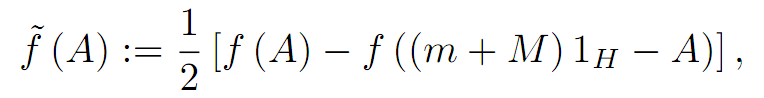where A is a selfadjoint operator in the Hilbert space H with the spectrum
Sp( A) ⊆ [ m,M] and f:[m,M] ->  C is a continuous function on [m,M]  are given. Applications for the power and logarithmic functions are provided as well.

2: Paper Source PDF document

Paper's Title:

On the Regularization of Hammerstein's type Operator Equations

Author(s):

E. Prempeh, I. Owusu-Mensah and K. Piesie-Frimpong

Department of Mathematics,
KNUST, Kumasi-
Ghana.

Department of Science Education,
University of Education,
Winneba

Department of Mathematics,
Presbyterian University College, Abetifi,
Ghana

E-mail: isaacowusumensah@gmail.com

E-mail: eprempeh.cos@knust.edu.gh

E-mail: piesie74@yahoo.com

Abstract:

We have studied Regularization of Hammerstein's Type Operator Equations in general Banach Spaces. In this paper, the results have been employed to establish regularized solutions to Hammerstein's type operator equations in Hilbert spaces by looking at three cases of regularization.

2: Paper Source PDF document

Paper's Title:

Hyponormal and K-Quasi-Hyponormal Operators On Semi-Hilbertian Spaces

Author(s):

Ould Ahmed Mahmoud Sid Ahmed and Abdelkader Benali

Mathematics Department,
College of Science,
Aljouf University,
Aljouf 2014,
Saudi Arabia.
E-mail: sididahmed@ju.edu.sa

Mathematics Department, Faculty of Science,
Hassiba Benbouali, University of Chlef,
B.P. 151 Hay Essalem, Chlef 02000,
Algeria.
E-mail: benali4848@gmail.com

Abstract:

Let H be a Hilbert space and let A be a positive bounded operator on H. The semi-inner product < u|v>A:=<Au|v>, u,v H induces a semi-norm || .||A on H. This makes H into a semi-Hilbertian space. In this paper we introduce the notions of hyponormalities and k-quasi-hyponormalities for operators on semi Hilbertian space (H,||.||A), based on the works that studied normal, isometry, unitary and partial isometries operators in these spaces. Also, we generalize some results which are already known for hyponormal and quasi-hyponormal operators. An operator T BA (H) is said to be (A, k)-quasi-hyponormal if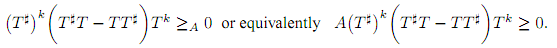2: Paper Source PDF document

Paper's Title:

The boundedness of Bessel-Riesz operators on generalized Morrey spaces

Author(s):

Mochammad Idris, Hendra Gunawan and Eridani

Department of Mathematics,
Bandung Institute of Technology,
Bandung 40132,
Indonesia.
E-mail: mochidris@students.itb.ac.id

Department of Mathematics,
Bandung Institute of Technology,
Bandung 40132,
Indonesia.
E-mail: hgunawan@math.itb.ac.id
URL: http://personal.fmipa.itb.ac.id/hgunawan/

Department of Mathematics,
Airlangga University,
Surabaya 60115,
Indonesia.

Abstract:

In this paper, we prove the boundedness of Bessel-Riesz operators on generalized Morrey spaces. The proof uses the usual dyadic decomposition, a Hedberg-type inequality for the operators, and the boundedness of Hardy-Littlewood maximal operator. Our results reveal that the norm of the operators is dominated by the norm of the kernels.

2: Paper Source PDF document

Paper's Title:

On The Rayleigh-Love Rod Accreting In Both Length And Cross-Sectional Area: Forced And Damped Vibrations

Author(s):

M.L.G. Lekalakala1, M. Shatalov2, I. Fedotov3, S.V. Joubert4

1Department of Mathematics, Vaal University of Technology, P.O. Box 1889, Secunda, 2302, South Africa.
E-mail1: glen@vut.ac.za

2,3,4Department of Mathematics and Statistics, Tshwane University of Technology, Pretoria, South Africa.

Abstract:

In this paper an elastic cylindrical rod that is subjected to forced and damped vibrations is considered. The rod is assumed to be isotropic. The applied external force of excitation is assumed to be harmonic, and the damping force is that of Kelvin-Voigt. The longitudinally vibrating rod is fixed at the left end and free at the other end. The rod is assumed to be accreting in length and cross-sectional area as it vibrates. The problem arising and the dynamics of the vibrating rod are described and investigated within the Rayleigh-Love theories of the rod. A partial differential equation describing the longitudinal displacement of the rod is formulated. The formulated partial differential equation, together with the corresponding boundary conditions as per the configuration of the rod, is solved numerically using the Galerkin-Kantorovich method. The frequency of vibration of the harmonic exciting force is kept constant in this investigation.

It is shown that in this periodically forced viscoelastic damped vibration, all the modes of vibration are subjected to the resonance behaviour within a proper time interval, depending on the length of the accreting rod.

2: Paper Source PDF document

Paper's Title:

Iterative Approximation of Zeros of Accretive Type Maps, with Applications

Author(s):

Charles Ejike Chidume, Chinedu Godwin Ezea, and Emmanuel Ezzaka Otubo

African University of Science and Technology, Abuja,
Nigeria.
E-mail: cchidume@aust.edu.ng
E-mail: chinedu.ezea@gmail.com
E-mail: mrzzaka@yahoo.com

Department of Mathematics,
Nnamdi Azikiwe University,
Awka,
Nigeria
E-mail: chinedu.ezea@gmail.com

Ebonyi State University,
Abakaliki,
Nigeria
E-mail: mrzzaka@yahoo.com

Abstract:

Let E be a reflexive real Banach space with uniformly Gâteaux differentiable norm. Let J:E E* be the normalized duality map on E and let A:E* E be a map such that AJ is an accretive and uniformly continuous map. Suppose that (AJ)-1(0) in nonempty. Then, an iterative sequence is constructed and proved to converge strongly to some u* in (AJ)-1(0). Application of our theorem in the case that E is a real Hilbert space yields a sequence which converges strongly to a zero of A. Finally, non-trivial examples of maps A for which AJ is accretive are presented..

2: Paper Source PDF document

Paper's Title:

Iterative Algorithm for Split Generalized Mixed Equilibrium Problem Involving Relaxed Monotone Mappings in Real Hilbert Spaces

Author(s):

1U.A. Osisiogu, F.L. Adum, and 2C. Izuchukwu

1Department of Mathematics and Computer Science,
Ebonyi State University, Abakaliki,
Nigeria.

2School of Mathematics, Statistics and Computer Science,
University of KwaZulu-Natal, Durban,
South Africa.
E-mail: izuchukwuc@ukzn.ac.za, izuchukwu_c@yahoo.com

Abstract:

The main purpose of this paper is to introduce a certain class of split generalized mixed equilibrium problem involving relaxed monotone mappings. To solve our proposed problem, we introduce an iterative algorithm and obtain its strong convergence to a solution of the split generalized mixed equilibrium problems in Hilbert spaces. As special cases of the proposed problem, we studied the proximal split feasibility problem and variational inclusion problem.

2: Paper Source PDF document

Paper's Title:

Solving Two Point Boundary Value Problems by Modified Sumudu Transform Homotopy Perturbation Method

Author(s):

Asem AL Nemrat and Zarita Zainuddin

School of Mathematical Sciences,
Universiti Sains Malaysia,
11800 Penang,
Malaysia.
E-mail: alnemrata@yahoo.com
zarita@usm.my

Abstract:

This paper considers a combined form of the Sumudu transform with the modified homotopy perturbation method (MHPM) to find approximate and analytical solutions for nonlinear two point boundary value problems. This method is called the modified Sumudu transform homotopy perturbation method (MSTHPM). The suggested technique avoids the round-off errors and finds the solution without any restrictive assumptions or discretization. We will introduce an appropriate initial approximation and furthermore, the residual error will be canceled in some points of the interval (RECP). Only a first order approximation of MSTHPM will be required, as compared to STHPM, which needs more iterations for the same cases of study. After comparing figures between approximate, MSTHPM, STHPM and numerical solutions, it is found through the solutions we have obtained that they are highly accurate, indicating that the MSTHPM is very effective, simple and can be used to solve other types of nonlinear boundary value problems (BVPs).

2: Paper Source PDF document

Paper's Title:

An Integration Technique for Evaluating Quadratic Harmonic Sums

Author(s):

J. M. Campbell and K.-W. Chen

Department of Mathematics and Statistics,
York University, 4700 Keele St, Toronto,
ON M3J 1P3,
E-mail: jmaxwellcampbell@gmail.com

Department of Mathematics, University of Taipei,
Taipei 10048, Taiwan.
E-mail: kwchen@uTaipei.edu.tw
URL: https://math.utaipei.edu.tw/p/412-1082-22.php

Abstract:

The modified Abel lemma on summation by parts has been applied in many ways recently to determine closed-form evaluations for infinite series involving generalized harmonic numbers with an upper parameter of two. We build upon such results using an integration technique that we apply to convert'' a given evaluation for such a series into an evaluation for a corresponding series involving squared harmonic numbers.

2: Paper Source PDF document

Paper's Title:

Several New Closed-form Evaluations of the Generalized Hypergeometric Function with Argument 1/16

Author(s):

B. R. Srivatsa Kumar, Insuk Kim and Arjun K. Rathie

Department of Mathematics,
Manipal Institute of Technology,
Manipal 576 104,
India.
E-mail: sri_vatsabr@yahoo.com

Department of Mathematics Education,
Wonkwang University,
Iksan, 54538,
Republic of Korea.
E-mail: iki@wku.ac.kr

Department of Mathematics,
Vedant College of Engineering and Technology,
Rajasthan Technical University,
Bundi, 323021, Rajasthan,
India.
E-mail: arjunkumarrathie@gmail.com

Abstract:

The main objective of this paper is to establish as many as thirty new closed-form evaluations of the generalized hypergeometric function q+1Fq(z) for q= 2, 3, 4. This is achieved by means of separating the generalized hypergeometric function q+1Fq(z) for q=1, 2, 3, 4, 5 into even and odd components together with the use of several known infinite series involving central binomial coefficients obtained earlier by Ji and Hei \& Ji and Zhang.

2: Paper Source PDF document

Paper's Title:

D-Iterative Method for Solving a Delay Differential Equation and a Two-Point Second-Order Boundary Value Problems in Banach Spaces

Author(s):

Francis Akutsah1, Akindele Adebayo Mebawondu2, Oluwatosin Babasola3, Paranjothi Pillay4 and Ojen Kumar Narain5

1School of Mathematics,
Statistics and Computer Science,
University of KwaZulu-Natal, Durban,
South Africa.
E-mail: 216040405@stu.ukzn.ac.za, akutsah@gmail.com

2School of Mathematics,
Statistics and Computer Science,
University of KwaZulu-Natal, Durban,
South Africa.
DST-NRF Centre of Excellence in Mathematical and Statistical Sciences (CoE-MaSS),
Johannesburg,
South Africa.
Mountain Top University,
Prayer City, Ogun State,
Nigeria.
E-mail: dele@aims.ac.za

3Department of Mathematical Sciences,
University of Bath,
Claverton Down,
Bath, BA2 7AY
UK.
E-mail: ob377@bath.ac.uk

4School of Mathematics,
Statistics and Computer Science,
University of KwaZulu-Natal, Durban,
South Africa.
E-mail: pillaypi@ukzn.ac.za

5School of Mathematics,
Statistics and Computer Science,
University of KwaZulu-Natal, Durban,
South Africa.
E-mail: naraino@ukzn.ac.za

Abstract:

The purpose of this paper is to re-establish the convergence, stability and data dependence results established by  and  by removing the strong assumptions imposed on the sequences which were used to obtain their results. In addition, we introduced a modified approach using the D-iterative method to solve a two-point second-order boundary value problem, and also obtain the solution of a delay differential equations using the obtained results in this paper. The results presented in this paper do not only extend and improve the results obtained in [2, 3], it further extends and improve some existing results in the literature.

1: Paper Source PDF document

Paper's Title:

Inequalities Relating to the Gamma Function

Author(s):

Chao-Ping Chen and Feng Qi

Department of Applied Mathematics and Informatics, Research Institute of Applied Mathematics,
Henan Polytechnic University, Jiaozuo City, Henan 454000, China

chenchaoping@hpu.edu.cn

Department of Applied Mathematics and Informatics, Research Institute of Applied Mathematics,
Henan Polytechnic University, Jiaozuo City, Henan 454000, China

qifeng@hpu.edu.cn, fengqi618@member.ams.org

U
rl: http://rgmia.vu.edu.au/qi.html, http://dami.hpu.edu.cn/qifeng.html

Abstract:

For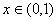, we have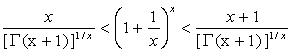.

For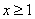,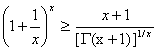,

And equality occurs for x=1.

1: Paper Source PDF document

Paper's Title:

Long Time Behavior for a Viscoelastic Problem with a Positive Definite Kernel

Author(s):

Nasser-eddine Tatar

King Fahd University of Petroleum and Mineral, Department of Mathematical Sciences,
Dhahran, 31261 Saudi Arabia

Abstract:

We study the asymptotic behavior of solutions for an integro-differential problem which arises in the theory of viscoelasticity. It is proved that solutions go to rest in an exponential manner under new assumptions on the relaxation function in the memory term. In particular, we consider a new family of kernels which are not necessarily decreasing.

1: Paper Source PDF document

Paper's Title:

An Application of Quasi Power Increasing Sequences

Author(s):

Hüseyín Bor

Department of Mathematics, Erciyes University, 38039 Kayseri, Turkey
rl:

Abstract:

In this paper a result of Bor  has been proved under weaker conditions by using a -quasi power increasing sequence instead of an almost increasing sequence.

1: Paper Source PDF document

Paper's Title:

Analytical and Numerical Solutions of the Inhomogenous Wave Equation

Author(s):

T. Matsuura and S. Saitoh

Department of Mechanical Engineering, Faculty of Engineering,
Gunma University, Kiryu 376-8515, Japan
matsuura@me.gunma-u.ac.jp

Department of Mathematics, Faculty of Engineering,
Gunma University, Kiryu 376-8515, Japan
ssaitoh@math.sci.gunma-u.ac.jp

Abstract:

In this paper, by a new concept and method we give approximate solutions of the inhomogenous wave equation on multidimensional spaces. Numerical experiments are conducted as well.

1: Paper Source PDF document

Paper's Title:

Multivalued Equilibrium Problems with Trifunction

Author(s):

Etisalat College of Engineering, P.O. Box 980, Sharjah, United Arab Emirates
noor@ece.ac.ae

Abstract:

In this paper, we use the auxiliary principle technique to suggest some new classes of iterative algorithms for solving multivalued equilibrium problems with trifunction. The convergence of the proposed methods either requires partially relaxed strongly monotonicity or pseudomonotonicity. As special cases, we obtain a number of known and new results for solving various classes of equilibrium and variational inequality problems. Since multivalued equilibrium problems with trifunction include equilibrium, variational inequality and complementarity problems as specials cases, our results continue to hold for these problems.

1: Paper Source PDF document

Paper's Title:

Generalized Fuglede-Putnam Theorem and Orthogonality

Author(s):

A. Bachir and A. Sagres

Department of Mathematics, Faculty of Science, King Khaled University, Abha, P.O. Box 9004 Kingdom Saudi Arabia

Abstract:

An asymmetric Fuglede-Putnam’s theorem for dominant operators and p-hyponormal operators is proved, as a consequence of this result, we obtain that the range of the generalized derivation induced by the above classes of operators is orthogonal to its kernel.

1: Paper Source PDF document

Paper's Title:

Monotonicity Properties for Generalized Logarithmic Means

Author(s):

Chao-Ping Chen and Feng Qi

Department of Applied Mathematics and Informatics, Research Institute of Applied Mathematics,
Henan Polytechnic University, Jiaozuo City, Henan 454000, China

chenchaoping@hpu.edu.cn

Department of Applied Mathematics and Informatics, Research Institute of Applied Mathematics,
Henan Polytechnic University, Jiaozuo City, Henan 454000, China

qifeng@hpu.edu.cn, fengqi618@member.ams.org

U
rl: http://rgmia.vu.edu.au/qi.html, http://dami.hpu.edu.cn/qifeng.html

Abstract:

In this paper, we consider the monotonicity properties for ratio of two generalized logarithmic means, and then use it to extend and complement a recently published result of F. Qi and B.-N. Guo.

1: Paper Source PDF document

Paper's Title:

A Majorization Problem for the Subclass of p-Valently Analytic Functions of Complex Order

Author(s):

Sezgin Akbulut

Department of Mathematics, Science and Art Faculty,
Atatürk University, 25240 Erzurum, Turkey
sbulut@atauni.edu.tr

Abstract:

The main purpose of this paper is to investigate a majorization problem for the class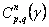. Relevant connections of the main result obtained in this paper with those given by earlier workers on the subject are also pointed out.

1: Paper Source PDF document

Paper's Title:

Reverses of the Triangle Inequality in Inner Product Spaces

Author(s):

Sever S. Dragomir

School of Computer Science and Mathematics,
Victoria University Of Technology,
PO Box 14428, Mcmc 8001,
Victoria, Australia.
sever@csm.vu.edu.au
Url: http://rgmia.vu.edu.au/SSDragomirWeb.html

Abstract:

Some new reverses for the generalised triangle inequality in inner product spaces are given. Applications in connection to the Schwarz inequality and for vector-valued integrals are provided as well.

1: Paper Source PDF document

Paper's Title:

Positive Solution For Discrete Three-Point Boundary Value Problems

Author(s):

Wing-Sum Cheung And Jingli Ren

Department of Mathematics,
The University of Hong Kong,
Pokfulam, Hong Kong
wscheung@hku.hk

Institute of Systems Science,
Beijing 100080, P.R. China
renjl@mx.amss.ac.cn

Abstract:

This paper is concerned with the existence of positive solution to the discrete three-point boundary value problem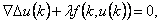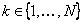,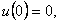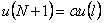where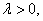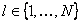, and f is allowed to change sign. By constructing available operators, we shall apply the method of lower solution and the method of topology degree to obtain positive solution of the above problem for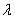on a suitable interval. The associated Green’s function is first given.

1: Paper Source PDF document

Paper's Title:

An alternative proof of monotonicity for the extended mean values

Author(s):

Chao-Ping Chen and Feng Qi

Department of Applied Mathematics and Informatics, Research Institute of Applied Mathematics,
Henan Polytechnic University, Jiaozuo City, Henan 454000, China

chenchaoping@hpu.edu.cn

Department of Applied Mathematics and Informatics, Research Institute of Applied Mathematics,
Henan Polytechnic University, Jiaozuo City, Henan 454000, China

qifeng@hpu.edu.cn, fengqi618@member.ams.org
Url
: http://rgmia.vu.edu.au/qi.html, http://dami.hpu.edu.cn/qifeng.html

Abstract:

An alternative proof of monotonicity for the extended mean values is given.

1: Paper Source PDF document

Paper's Title:

Generalizations of two theorems on absolute summability methods

Author(s):

H.S. Özarslan and H.N. Öğdük

Department of Mathematics,
Erciyes University, 38039 Kayseri,
Turkey
seyhan@erciyes.edu.tr
nogduk@erciyes.edu.tr
URL: http://fef.erciyes.edu.tr/math/hikmet.htm

Abstract:

In this paper two theorems on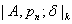summability methods, which generalize two theorems of Bor on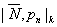summability methods, have been proved.

1: Paper Source PDF document

Paper's Title:

Reverse of Martin's Inequality

Author(s):

Chao-Ping Chen, Feng Qi, and Sever S. Dragomir

Department of Applied Mathematics and Informatics,
Research Institute of Applied Mathematics,
Henan Polytechnic University,
Jiaozuo City, Henan 454010, China
chenchaoping@sohu.com; chenchaoping@hpu.edu.cn

Department of Applied Mathematics and Informatics,
Research Institute of Applied Mathematics,
Henan Polytechnic University,
Jiaozuo City, Henan 454010, China
qifeng@hpu.edu.cn
Url: http://rgmia.vu.edu.au/qi.html

School of Computer Science and Mathematics,
Victoria University of Technology,
P. O. Box 14428, Melbourne City Mc,
Victoria 8001, Australia
Sever.Dragomir@vu.edu.au
Url: http://rgmia.vu.edu.au/SSDragomirWeb.html

Abstract:

In this paper, it is proved that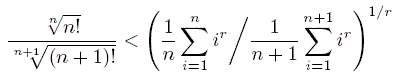for all natural numbers n, and all real r < 0.

1: Paper Source PDF document

Paper's Title:

On Certain Classes of Harmonic Univalent Functions Based on Salagean Operator

Author(s):

G. Murugusundaramoorthy, Thomas Rosy, and B. A. Stephen

Department of Applied Mathematics and Informatics,
Department of Mathematics, Vellore Institute of Technology,
Deemed University, Vellore - 632014, India.
gmsmoorthy@yahoo.com

Department of Applied Mathematics and Informatics,
Department of Mathematics, Madras Christian College,
Chennai - 600059, India.
drthomasrosy@rediffmail.com

Abstract:

We define and investigate a class of complex-valued harmonic univalent functions of the form f = h + g using Salagean operator where h and g are analytic in the unit disc U = { z : |z| < 1 }. A necessary and sufficient coefficient conditions are given for functions in these classes. Furthermore, distortion theorems, inclusion relations, extreme points, convolution conditions and convex combinations for this family of harmonic functions are obtained.

1: Paper Source PDF document

Paper's Title:

Compactly Supported Interpolatory Orthogonal Multiwavelet Packets

Author(s):

Yang Shouzhi

Department of Mathematics,
Shantou University,
Shantou, Po Box 515063,
P.R.China.
szyang@stu.edu.cn

Abstract:

Compactly supported interpolatory orthogonal multiwavelet packets are introduced. Precisely, if both the multiscaling function and the corresponding multiwavelet have the same interpolatory property, then the multiwavelet packets are also interpolatory orthogonal. Thus, the coefficients of decomposition or synthesis of multiwavelet packets can be realized by sampling instead of inner products. This multiwavelet packets provide a finer decomposition of multiwavelet packets space and give a better localization.

1: Paper Source PDF document

Paper's Title:

General Oscillations for Some Third Order Differential Systems with Nonlinear Acceleration Term

Author(s):

Awar Simon Ukpera

Department of Mathematics,
Obafemi Awolowo University,
Ile-Ife,
Nigeria.
aukpera@oauife.edu.ng

Abstract:

We generate some general nonuniform hypotheses for third order differential systems of the form X''' +F(t,X'' )+BX'+CX = P(t), in which B and C are not necessarily constant matrices. Some results requiring sharp conditions on this system have recently been published by the author in . This work however examines more closely crucial properties associated with the generalised nature of the nonlinear acceleration term F, which were largely overlooked in the earlier paper.

1: Paper Source PDF document

Paper's Title:

On the Hohov Convolution Of The Class Sp(α,β)

Author(s):

T. N. Shanmugam and S. Sivasubramanian

Department of Mathematics,
Anna University,
Chennai 600025,
shan@annauniv.edu

Department of Mathematics,
Easwari Engineering College,
Chennai-600089,
sivasaisastha@rediffmail.com

Abstract:

Let F(a,b;c;z) be the Gaussian hypergeometric function and Ia,b;c(f)=zF(a,b;c;z)*f(z) be the Hohlov operator defined on the class A of all normalized analytic functions. We determine conditions on the parameters a,b,c such that Ia,b;c(f) will be in the class of parabolic starlike functions Sp(α,β). Our results extend several earlier results.

1: Paper Source PDF document

Paper's Title:

Logarithmically complete monotonicity properties for the gamma functions

Author(s):

Chao-Ping Chen and Feng Qi

Department of Applied Mathematics and Informatics,
Research Institute of Applied Mathematics,
Henan Polytechnic University,
Jiaozuo City, Henan 454010,
China.
chenchaoping@hpu.edu.cn

Department of Applied Mathematics and Informatics,
Research Institute of Applied Mathematics,
Henan Polytechnic University,
Jiaozuo City, Henan 454010,
China.
qifeng@hpu.edu.cn
fengqi618@member.ams.org

Abstract:

Some logarithmically completely monotonic functions involving the gamma functions are presented. As a consequence, some known results are proved and refined.

1: Paper Source PDF document

Paper's Title:

Boundedness for Vector-Valued Multilinear Singular Integral Operators on Triebel-Lizorkin Spaces

Author(s):

Liu Lanzhe

College of Mathematics
Changsha University of Science and Technology,
Changsha 410077,
P.R. of China.
lanzheliu@263.net

Abstract:

In this paper, the boundedness for some vector-valued multilinear operators associated to certain fractional singular integral operators on Triebel-Lizorkin space are obtained. The operators include Calderón-Zygmund singular integral operator and fractional integral operator.

1: Paper Source PDF document

Paper's Title:

Stability of a Pexiderized Equation

Author(s):

Maryam Amyar

Department of Mathematics,
and Banach Mathematical Research Group (BMRG)
amyari@mshdiau.ac.ir

URL: http://amyari.mshdiau.ac.ir

Abstract:

The aim of the paper is to prove the stability of the Pexiderized equation f(x)=g(y+x)-h(y-x), for any amenable abelian group.

1: Paper Source PDF document

Paper's Title:

Boundary Value Problems for Fractional Diffusion-Wave equation

Author(s):

Varsha Daftardar-Gejji and Hossein Jafari

Department of Mathematics, University of Pune,
Ganeshkhind, Pune - 411007,
INDIA.
vsgejji@math.unipune.ernet.in
jafari_h@math.com

Abstract:

Non homogeneous fractional diffusion-wave equation has been solved under linear/nonlinear boundary conditions. As the order of time derivative changes from 0 to 2, the process changes from slow diffusion to classical diffusion to mixed diffusion-wave behaviour.
Numerical examples presented here confirm this inference. Orthogonality of eigenfunctions in case of fractional Stürm-Liouville problem has been established

1: Paper Source PDF document

Paper's Title:

Merit Functions and Error Bounds for Mixed Quasivariational Inequalities

Author(s):

Mathematics Department, COMSATS Institute of Information Technology,
noormaslam@hotmail.com

Abstract:

It is well known that the mixed quasivariational inequalities are equivalent to the fixed point problems. We use this equivalent alternative formulation to construct some merit functions for mixed quasivariational inequalities and obtain error bounds under some conditions. Since mixed quasivariational inequalities include the classical variational inequalities and the complementarity problems as special cases, our results continue to hold for these problems.

1: Paper Source PDF document

Paper's Title:

A New Family of Periodic Functions as Explicit Roots of a Class of Polynomial Equations

Author(s):

M. Artzrouni

Department of Mathematics, University of Pau
64013 Pau Cedex
Pau, France
marc.artzrouni@univ-pau.fr
URL: http://www.univ-pau.fr/~artzroun

Abstract:

For any positive integer n a new family of periodic functions in power series form and of period n is used to solve in closed form a class of polynomial equations of order n. The n roots are the values of the appropriate function from that family taken at 0, 1, ... , n-1.

1: Paper Source PDF document

Paper's Title:

Some Stability Results For Fixed Point Iteration Processes

Author(s):

M. O. Olatinwo, O. O. Owojori, and C. O. Imoru

Department of Mathematics, Obafemi Awolowo University,
Ile-Ife,
Nigeria.
polatinwo@oauife.edu.ng
walejori@oauife.edu.ng
cimoru@oauife.edu.ng

Abstract:

In this paper, we present some stability results for both the general Krasnoselskij and the Kirk's iteration processes. The method of Berinde \cite{VBE1} is employed but a more general contractive condition than those of Berinde \cite{VBE1}, Harder and Hicks \cite{HAM}, Rhoades \cite{RHO1} and Osilike \cite{OSI1} is considered.

1: Paper Source PDF document

Paper's Title:

Comparison Results for Solutions of Time Scale Matrix Riccati Equations and Inequalities

Author(s):

R. Hilscher

Department of Mathematical Analysis, Faculty of Science,
Masaryk University, Jan
áčkovo nám. 2a,
CZ-60200, Brno, Czech Republic.
hilscher@math.muni.cz
URL: http://www.math.muni.cz/~hilscher/

Abstract:

In this paper we derive comparison results for Hermitian solutions of time scale matrix Riccati equations and Riccati inequalities. Such solutions arise from special conjoined bases (X,U) of the corresponding time scale symplectic system via the Riccati quotient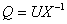. We also discuss properties of a unitary matrix solution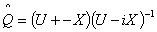of a certain associated Riccati equation.

1: Paper Source PDF document

Paper's Title:

A Strengthened Hardy-Hilbert's Type Inequality

Author(s):

Weihong Wang and Bicheng Yang

Department of Mathematics, Guangdong Education Institute,
Guangzhou, Guangdong 520303,
People's Republic Of China
wwh@gdei.edu.cn
bcyang@pub.guangzhou.gd.cn
URL: http://www1.gdei.edu.cn/yangbicheng/index.html

Abstract:

By using the improved Euler-Maclaurin's summation formula and estimating the weight coefficient, we give a new strengthened version of the more accurate Hardy-Hilbert's type inequality. As applications, a strengthened version of the equivalent form is considered.

1: Paper Source PDF document

Paper's Title:

On Positive Entire Solutions of Second Order Quasilinear Elliptic Equations

Author(s):

Zuodong Yang and Honghui Yin

Institute of Mathematics, School of Mathematics and Computer Science,
Nanjing Normal University, Jiangsu Nanjing 210097,
China;
zdyang_jin@263.net

Department of Mathematics, Huaiyin Teachers College,
Jiangsu Huaian 223001,
China;
School of Mathematics and Computer Sciences,
Nanjing Normal University, Jiangsu Nanjing 210097,
China.
yin_hh@sina.com

Abstract:

In this paper, our main purpose is to establish the existence theorem of positive entire solutions of second order quasilinear elliptic equations under new conditions. The main results of the present paper are new and extend the previously known results.

1: Paper Source PDF document

Paper's Title:

Numerical Studies on Dynamical Systems Method for Solving Ill-posed Problems with Noise

Author(s):

N. H. Sweilam and A. M. Nagy

Mathematics Department, Faculty of Science,
Cairo University,
Giza, Egypt.
n_sweilam@yahoo.com

Mathematics Department, Faculty of Science,
Benha University,
Benha, Egypt.
abdelhameed_nagy@yahoo.com

Abstract:

In this paper, we apply the dynamical systems method proposed by A. G. RAMM, and the the variational regularization method to obtain numerical solution to some ill-posed problems with noise. The results obtained are compared to exact solutions. It is found that the dynamical systems method is preferable because it is easier to apply, highly stable, robust, and it always converges to the solution even for large size models.

1: Paper Source PDF document

Paper's Title:

A New Step Size Rule in Noor's Method for Solving General Variational Inequalities

Author(s):

Abdellah Bnouhachem

School of Management Science and Engineering, Nanjing University,
Nanjing, 210093
P.R. China.
babedallah@yahoo.com

Abstract:

In this paper, we propose a new step size rule in Noor's method for solving general variational inequalities. Under suitable conditions, we prove that the new method is globally convergent. Preliminary numerical experiments are included to illustrate the advantage and efficiency of the proposed method.

1: Paper Source PDF document

Paper's Title:

Generalized Quasilinearization Method for the Forced Düffing Equation

Author(s):

Ramzi S. N. Alsaedi

Department of Mathematics, King Abdul Aziz University,
Jeddah P.O. Box 80203,
Saudi Arabia.
ramzialsaedi@yahoo.co.uk

Abstract:

A generalized quasilinearization method for the periodic problem related to the forced D\"{u}ffing equation is developed and a sequence of approximate solutions converging monotonically and quadratically to the solution of the given problem is presented.

1: Paper Source PDF document

Paper's Title:

Iterated Order of Fast Growth Solutions of Linear Differential Equations

Author(s):

Benharrat Belaďdi

Department of Mathematics
Laboratory of Pure and Applied Mathematics
University of Mostaganem
B. P. 227 Mostaganem,
ALGERIA.
belaidi@univ-mosta.dz

Abstract:

In this paper, we investigate the growth of solutions of the differential equation f(k) + Ak-1 (z) f(k-1) +...+ A1 (z) f' + A0 (z) f= F (z), where Ao (z), ..., Ak-1 (z) and F (z)0 are entire functions. Some estimates are given for the iterated order of solutions of the above quation when one of the coefficients As is being dominant in the sense that it has larger growth than Aj (j≠s) and F.

1: Paper Source PDF document

Paper's Title:

Existence of Solutions for Third Order Nonlinear Boundary Value Problems

Author(s):

Yue Hu and Zuodong Yang

School of Mathematics and Computer Science, Nanjing Normal University, Jiangsu Nanjing 210097,
China.
huu3y2@163.com

College of Zhongbei, Nanjing Normal University, Jiangsu Nanjing 210046,
China.
zdyang_jin@263.net
yangzuodong@njnu.edu.cn

Abstract:

In this paper, the existence of solution for a class of third order quasilinear ordinary differential equations with nonlinear boundary value problems

p(u"))'=f(t,u,u',u"), u(0)=A, u'(0)=B, R(u'(1),u"(1))=0

is established. The results are obtained by using upper and lower solution methods.

1: Paper Source PDF document

Paper's Title:

On Sandwich Theorems for Certain Subclass of Analytic Functions Involving Dziok-Srivastava Operator

Author(s):

T. N. Shanmugam, M. P. Jeyarama and A. Singaravelu

Department of Mathematics
College of Engineering, Anna University
Chennai - 600 025,
India
drtns2001@yahoo.com

Department of Mathematics
Easwari Engineering College
Ramapuram, Chennai - 600089
jeyaraman-mp@yahoo.co.i

Department of Mathematics
Valliammai Engineering College
Chennai - 603203
asing-59@yahoo.com

Abstract:

The purpose of this present paper is to derive some subordination and superordination results for certain normalized analytic functions in the open unit disk, acted upon by Dziok-Srivastava operator. Relevant connections of the results, which are presented in this paper, with various known results are also considered.

1: Paper Source PDF document

Paper's Title:

An Approximation of Jordan Decomposable Functions for a Lipschitz Function

Author(s):

Ibraheem Alolyan

Mathematics Department,
College of Science, King Saud University
Saudi Arabia
ialolyan05@yahoo.com

Abstract:

The well known Jordan decomposition theorem gives the useful characterization that any function of bounded variation can be written as the difference of two increasing functions. Functions which can be expressed in this way can be used to formulate an exclusion test for the recent Cellular Exclusion Algorithms for numerically computing all zero points or the global minima of functions in a given cellular domain [2,8,9]. In this paper we give an algorithm to approximate such increasing functions when only the values of the function of bounded variation can be computed. For this purpose, we are led to introduce the idea of ε-increasing functions. It is shown that for any Lipschitz continuous function, we can find two ε-increasing functions such that the Lipschitz function can be written as the difference of these functions.

1: Paper Source PDF document

Paper's Title:

On a Subclass of Uniformly Convex Functions Defined by the Dziok-Srivastava Operator

Author(s):

M. K. Aouf and G. Murugusundaramoorthy

Mathematics Department, Faculty of Science,
Mansoura University 35516,
Egypt.
mkaouf127@yahoo.com

School of Science and Humanities, VIT University
Vellore - 632014,
India.
gmsmoorthy@yahoo.com

Abstract:

Making use of the Dziok-Srivastava operator, we define a new subclass Tlm([α1];α,β) of uniformly convex function with negative coefficients. In this paper, we obtain coefficient estimates, distortion theorems, locate extreme points and obtain radii of close-to-convexity, starlikeness and convexity for functions belonging to the class Tlm([α1];α,β) . We consider integral operators associated with functions belonging to the class Hlm([α1];α,β) defined via the Dziok-Srivastava operator. We also obtain several results for the modified Hadamard products of functions belonging to the class Tlm([α1];α,β) and we obtain properties associated with generalized fractional calculus operators.

1: Paper Source PDF document

Paper's Title:

Positive Solutions for Systems of Three-point Nonlinear Boundary Value Problems

Author(s):

J. Henderson and S. K. Ntouyas

Department of Mathematics, Baylor University
Waco, Texas
76798-7328 USA.
Johnny_Henderson@baylor.edu
URL: http://www3.baylor.edu/~Johnny_Henderson

Department of Mathematics, University of Ioannina
451 10 Ioannina,
Greece.
sntouyas@cc.uoi.gr
URL: http://www.math.uoi.gr/~sntouyas

Abstract:

Values of λ are determined for which there exist positive solutions of the system of three-point boundary value problems, u''(t)+ λa(t)f(v(t))=0, v''(t)+λb(t)g(u(t))=0, for 0 < t <1, and satisfying, u(0) = 0, u(1)=α u(η), v(0) = 0, v(1)=α v(η). A Guo-Krasnosel'skii fixed point theorem is applied.

1: Paper Source PDF document

Paper's Title:

On Some Remarkable Product of Theta-function

Author(s):

M. S. Mahadeva Naika, M. C. Maheshkumar and K. Sushan Bairy

Department of Mathematics,
Bangalore University, Central College Campus,
Bangalore-560 001,
INDIA
msmnaika@rediffmail.com
softmahe@rediffmail.com
ksbairy@gmail.com

Abstract:

On pages 338 and 339 in his first notebook, Ramanujan records eighteen values for a certain product of theta-function. All these have been proved by B. C. Berndt, H. H. Chan and L-C. Zhang . Recently M. S. Mahadeva Naika and B. N. Dharmendra [7, 8] and Mahadeva Naika and M. C. Maheshkumar  have obtained general theorems to establish explicit evaluations of Ramanujan's remarkable product of theta-function. Following Ramanujan we define a new function bM,N as defined in (1.5). The main purpose of this paper is to establish some new general theorems for explicit evaluations of product of theta-function.

1: Paper Source PDF document

Paper's Title:

Some Inequalities for a Certain Class of Multivalent Functions Using Multiplier Transformation

Author(s):

Department Of Applied Mathematics
Sri Venkateswara College Of Engineering
Sriperumbudur, Chennai - 602105,
India.
suchithravenkat@yahoo.co.in

Department Of Mathematics,
Chennai - 600059,
India.

Department Of Applied Mathematics
Sri Venkateswara College Of Engineering
Sriperumbudur, Chennai - 602105,
India.
ganga@svce.ac.in

Department Of Mathematics,
Easwari Engineering College
Ramapuram, Chennai - 600089,
India.
ganga@svce.ac.in

Abstract:

The object of the present paper is to derive several inequalities associated with differential subordinations between analytic functions and a linear operator defined for a certain family of p-valent functions, which is introduced here by means of a family of extended multiplier transformations. Some special cases and consequences of the main results are also considered.

1: Paper Source PDF document

Paper's Title:

The Bernoulli Inequality in Uniformly Complete f-algebras with Identity

Author(s):

Adel Toumi and Mohamed Ali Toumi

Laboratoire de Physique des Liquides Critiques, Département de Physique, Faculté des Sciences de Bizerte, 7021, Zarzouna, Bizerte, TUNISIA

Département de Mathématiques, Faculté des Sciences de Bizerte, 7021, Zarzouna, Bizerte, TUNISIA
MohamedAli.Toumi@fsb.rnu.tn

Abstract:

The main purpose of this paper is to establish with a constructive proof the Bernoulli inequality: let A be a uniformly complete f-algebra with e as unit element, let 1<p<∞, then

(e+a)p≥e+pa

for all a∈A+. As an application we prove the Hölder inequality for positive linear functionals on a uniformly complete f-algebra with identity by using the Minkowski inequality.

1: Paper Source PDF document

Paper's Title:

Error Inequalities for Weighted Integration Formulae and Applications

Author(s):

Department of Mathematics
University of Split
Teslina 12/III, 21000 Split
CROATIA.
ujevic@pmfst.hr
ivalek@pmfst.hr

Abstract:

Weighted integration formulae are derived. Error inequalities for the weighted integration formulae are obtained. Applications to some special functions are also given.

1: Paper Source PDF document

Paper's Title:

Some Generalized Difference Sequence Spaces Defined by Orlicz Functions

Author(s):

Ramzi S. N. Alsaedi and Ahmad H. A. Bataineh

Department of Mathematics, King Abdul Aziz University,
Jeddah P.O.Box 80203,
Saudia Arabia
ramzialsaedi@yahoo.co.uk

Department of Mathematics, Al al-Bayt University,
Mafraq 25113,
Jordan
ahabf2003@yahoo.ca

Abstract:

In this paper, we define the sequence spaces: [V,M,p,u,Δ ],[V,M,p,u,Δ]0 and [V,M,p,u,Δ], where for any sequence x=(xn), the difference sequence Δx is given by Δx=(Δxn)= (xn-xn-1). We also study some properties and theorems of these spaces. These are generalizations of those defined and studied by Savas and Savas and some others before.

1: Paper Source PDF document

Paper's Title:

Regular Variation on Time Scales and Dynamic Equations

Author(s):

Pavel Řehák

Institute of Mathematics, Academy of Sciences of the Czech Republic
Žižkova 22, CZ61662 Brno,
Czech Republic
rehak@math.muni.cz
URL:http://www.math.muni.cz/~rehak

Abstract:

The purpose of this paper is twofold. First, we want to initiate a study of regular variation on time scales by introducing this concept in such a way that it unifies and extends well studied continuous and discrete cases. Some basic properties of regularly varying functions on time scales will be established as well. Second, we give conditions under which certain solutions of linear second order dynamic equations are regularly varying. Open problems and possible directions for a future research are discussed, too.

1: Paper Source PDF document

Paper's Title:

Contact With Adhesion between a Deformable Body and a Foundation

Author(s):

B. Teniou and M. Sofonea

Laboratoire de Mathematiques Appliquées et Modélisation,
Université Mentouri, Constantine 25000,
Algeria
tenioubou2@yahoo.fr

Laboratoire de Mathématiques et Physiques pour les Systémes,
Univesité de Perpignan,
France.
sofonea@univ-perp.fr

Abstract:

The aim of this work is study a dynamic contact problem between a deformable body and a foundation where the deformations are supposed to be small. The contact is with adhesion and normal compliance. The behavior of this body is modeled by a nonlinear elastic-visco-plastic law. The evolution of bonding field is described by a nonlinear differential equation. We derive a variational formulation of the contact problem and we prove the existence and uniqueness of its solution. The proof is based on the construction of three intermediate problems and then we construct a contraction mapping whose unique fixed point will be the weak solution of the mechanical problem.

1: Paper Source PDF document

Paper's Title:

Some Properties of the Solution of a Second Order Elliptic Abstract Differential Equation

Author(s):

A. Aibeche and K. Laidoune

Mathematics Department, Faculty of Sciences, University Ferhat Abbas, Setif,
Route de Scipion, 19000, Setif,
Algeria
aibeche@univ-setif.dz

Abstract:

In this paper we study a class of non regular boundary value problems for elliptic differential-operator equation of second order with an operator in boundary conditions. We give conditions which guarantee the coerciveness of the solution of the considered problem, the completeness of system of root vectors in Banach-valued functions spaces and we establish the Abel basis property of this system in Hilbert spaces. Finally, we apply this abstract results to a partial differential equation in cylindrical domain.

1: Paper Source PDF document

Paper's Title:

A Method for Solving Systems of Nonlinear Equations

Author(s):

J. Shokri

Department of Mathematics, Urmia University,
P. O. Box 165, Urmia,
Iran
j.shokri@urmia.ac.ir

Abstract:

In this paper, we suggest and analyze a new two-step iterative method for solving nonlinear equation systems using the combination of midpoint quadrature rule and Trapezoidal quadrature rule. We prove that this method has quadratic convergence. Several examples are given to illustrate the efficiency of the proposed method.

1: Paper Source PDF document

Paper's Title:

On ε-simultaneous Approximation in Quotient Spaces

Author(s):

H. Alizadeh, Sh. Rezapour, S. M. Vaezpour

Islamic University, Science and Research Branch, Tehran,
Iran

Department of Mathematics, Azarbaidjan
University of Tarbiat Moallem, Tabriz,
Iran

Department of Mathematics, Amirkabir
University of Technology, Tehran,
Iran

sh.rezapour@azaruniv.edu
vaez@aut.ac.ir

URL:http://www.azaruniv.edu/~rezapour
URL:http://math-cs.aut.ac.ir/vaezpour

Abstract:

The purpose of this paper is to develop a theory of best simultaneous approximation to ε-simultaneous approximation. We shall introduce the concept of ε-simultaneous pseudo Chebyshev, ε-simultaneous quasi Chebyshev and ε-simultaneous weakly Chebyshev subspaces of a Banach space. Then, it will be determined under what conditions these subspaces are transmitted to and from quotient spaces.

1: Paper Source PDF document

Paper's Title:

A Double Inequality for Divided Differences and Some Identities of the Psi and Polygamma Functions

Author(s):

B. N. Guo and F. Qi

School of Mathematics and Informatics, Henan Polytechnic University, Jiaozuo City, Henan Province, 454010, China
bai.ni.guo@gmail.com, bai.ni.guo@hotmail.com

School of Research Institute of Mathematical Inequality Theory, Henan Polytechnic University, Jiaozuo City, Henan Province, 454010, China
qifeng618@gmail.com, qifeng618@hotmail.com, qifeng618@qq.com
URL:http://qifeng618.spaces.live.com

Abstract:

In this short note, from the logarithmically completely monotonic property of the function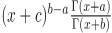, a double inequality for the divided differences and some identities of the psi and polygamma functions are presented.

1: Paper Source PDF document

Paper's Title:

On a Method of Proving the Hyers-Ulam Stability of Functional Equations on Restricted Domains

Author(s):

Janusz Brzdęk

Department of Mathematics
Pedagogical University Podchor
ąźych 2,
30-084 Krak
ów,
Poland
jbrzdek@ap.krakow.pl

Abstract:

We show that generalizations of some (classical) results on the Hyers-Ulam stability of functional equations, in several variables, can be very easily derived from a simple result on stability of a functional equation in single variable

1: Paper Source PDF document

Paper's Title:

Differentiability of Distance Functions in p-Normed Spaces

Author(s):

M. S. Moslehian, A. Niknam, S. Shadkam Torbati

Department of Pure Mathematics, Centre of Excellence in Analysis on Algebraic Structures (CEAAS),,
Iran

moslehian@ferdowsi.um.ac.ir
niknam@math.um.ac.ir

Abstract:

The farthest point mapping in a p-normed space X is studied in virtue of the Gateaux derivative and the Frechet derivative. Let M be a closed bounded subset of X having the uniformly p-Gateaux differentiable norm. Under certain conditions, it is shown that every maximizing sequence is convergent, moreover, if M is a uniquely remotal set then the farthest point mapping is continuous and so M is singleton. In addition, a Hahn--Banach type theorem in $p$-normed spaces is proved.

1: Paper Source PDF document

Paper's Title:

Fixed Points and Stability of the Cauchy Functional Equation

Author(s):

Choonkil Park and Themistocles M. Rassias

Department of Mathematics, Hanyang University,
Seoul 133-791,
Republic of Korea

Department of Mathematics, National Technical University of Athens,
Zografou Campus, 15780 Athens,
Greece

baak@hanyang.ac.kr
trassias@math.ntua.gr

Abstract:

Using fixed point methods, we prove the generalized Hyers-Ulam stability of homomorphisms in Banach algebras and of derivations on Banach algebras for the Cauchy functional equation.

1: Paper Source PDF document

Paper's Title:

Hyperbolic Barycentric Coordinates

Author(s):

Abraham A. Ungar

Department of Mathematics, North Dakota State University,
Fargo, ND 58105,
USA
Abraham.Ungar@ndsu.edu
URL
: http://math.ndsu.nodak.edu/faculty/ungar/

Abstract:

A powerful and novel way to study Einstein's special theory of relativity and its underlying geometry, the hyperbolic geometry of Bolyai and Lobachevsky, by analogies with classical mechanics and its underlying Euclidean geometry is demonstrated. The demonstration sets the stage for the extension of the notion of barycentric coordinates in Euclidean geometry, first conceived by Möbius in 1827, into hyperbolic geometry. As an example for the application of hyperbolic barycentric coordinates, the hyperbolic midpoint of any hyperbolic segment, and the centroid and orthocenter of any hyperbolic triangle are determined.

1: Paper Source PDF document

Paper's Title:

A Study of the Effect of Density Dependence in a Matrix Population Model

Author(s):

N. Carter and M. Predescu

Department of Mathematical Sciences,
Bentley University,
Waltham, MA 02452,
U.S.A.
ncarter@bentley.edu
mpredescu@bentley.edu

Abstract:

We study the behavior of solutions of a three dimensional discrete time nonlinear matrix population model. We prove results concerning the existence of equilibrium points, boundedness, permanence of solutions, and global stability in special cases of interest. Moreover, numerical simulations are used to compare the dynamics of two main forms of the density dependence function (rational and exponential).

1: Paper Source PDF document

Paper's Title:

Inclusion and Neighborhood Properties for Certain Subclasses of Analytic Functions Associated with Convolution Structure

Author(s):

M. K. Aouf

Mathematics Department,
Faculty of Science,
Mansoura University 35516,
Egypt.
mkaouf127@yahoo.com

Abstract:

In this paper we introduce and investigate two new subclasses of multivalently analytic functions of complex order defined by using the familiar convolution structure of analytic functions. In this paper we obtain the coefficient estimates and the consequent inclusion relationships involving the neighborhoods of the p-valently analytic functions.

1: Paper Source PDF document

Paper's Title:

On the Fock Representation of the Central Extensions of the Heisenberg Algebra

Author(s):

L. Accardi and A. Boukas

Centro Vito Volterra, Universitŕ di Roma Tor Vergata,
via Columbia 2, 00133 Roma,
Italy
accardi@volterra.mat.uniroma2.it
URL: http://volterra.mat.uniroma2.it

Department of Mathematics,
American College of Greece,
Greece
andreasboukas@acg.edu

Abstract:

We examine the possibility of a direct Fock representation of the recently obtained non-trivial central extensions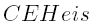of the Heisenberg algebra, generated by elements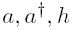and E satisfying the commutation relations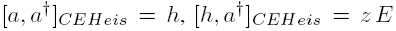,  and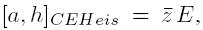, where a and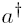are dual, h is self-adjoint, E is the non-zero self-adjoint central element and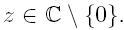We define the exponential vectors associated with theFock space, we compute their Leibniz function (inner product), we describe the action of a,and h on the exponential vectors and we compute the moment generating and characteristic functions of the classical random variable corresponding to the self-adjoint operator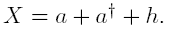1: Paper Source PDF document

Paper's Title:

On the product of M-measures in l-groups

Author(s):

A. Boccuto, B. Riěcan, and A. R. Sambucini

Dipartimento di Matematica e Informatica,
via Vanvitelli, 1 I-06123 Perugia,
Italy.
boccuto@dipmat.unipg.it
URL: http://www.dipmat.unipg.it/~boccuto

Katedra Matematiky, Fakulta Prírodných Vied,
Univerzita Mateja Bela,
Tajovského, 40, Sk-97401 Banská Bystrica,
Slovakia.
riecan@fpv.umb.sk

Dipartimento di Matematica e Informatica,
via Vanvitelli, 1 I-06123 Perugia,
Italy.
matears1@unipg.it
URL: http://www.unipg.it/~matears1

Abstract:

Some extension-type theorems and compactness properties for the
product of l-group-valued M-measures are proved.

1: Paper Source PDF document

Paper's Title:

Asymptotic Distribution of Products of Weighted Sums of Dependent Random Variables

Author(s):

Y. Miao and J. F. Li

College of Mathematics and Information Science,
Henan Normal University
Henan, China
yumiao728@yahoo.com.cn

College of Mathematics and Information Science,
Henan Normal University, 453007
Henan, China.
junfen_li@yahoo.com.cn

Abstract:

In this paper we establish the asymptotic distribution of products of weighted sums of dependent positive random variable, which extends the results of Rempała and Wesołowski (2002).

1: Paper Source PDF document

Paper's Title:

On a Class of Uniformly Convex Functions Defined by Convolution with Fixed Coefficient

Author(s):

T. N. Shanmugam, S. Sivasubramanian, and G. Murugusundaramoorthy

Department of Mathematics,
College of Engineering,
Anna University,
Chennai - 600 025,
India.

drtns2001@yahoo.com

Department of Mathematics,
University College of Engineering,
Tindivanam
Anna University-Chennai,
Saram-604 703,
India.
sivasaisastha@rediffmail.com

School of Sciences
and Humanities,
VIT University, Vellore-632 014,
India.
gmsmoorthy@yahoo.com

Abstract:

We define a new subclass of uniformly convex functions with negative and fixed second coefficients defined by convolution. The main object of this paper is to obtain coefficient estimates distortion bounds, closure theorems and extreme points for functions belong to this new class . The results are generalized to families with fixed finitely many coefficients.

1: Paper Source PDF document

Paper's Title:

Real Interpolation Methods and Quasilogarithmic Operators

Author(s):

Ming Fan

School of Industrial Technology and Management,
Dalarna University, 781 88 Borlänge, Sweden

fmi@du.se
URL: http://users.du.se/~fmi

Abstract:

The purpose of this paper is to deal with nonlinear quasilogarithmic operators, which possesses the uniformly bounded commutator property on various interpolation spaces in the sense of Brudnyi-Krugljak associated with the quasi-power parameter spaces. The duality, and the domain and range spaces of these operators are under consideration. Some known inequalities for the Lebesgue integration spaces and the trace classes are carried over to the non-commutative symmetric spaces of measurable operators affiliated with a semi-finite von Neumann algebra.

1: Paper Source PDF document

Paper's Title:

Solution of One Conjecture on Inequalities with Power-Exponential Functions.

Author(s):

Seiichi Manyama

Osaka University

Japan

manchanr4@gmail.com

Abstract:

In this paper, we prove the open inequality aea+beb aeb+bea for all positive real numbers a and b.

1: Paper Source PDF document

Paper's Title:

Topological Aspects of Scalarization in Vector Optimization Problems.

Author(s):

Peter I. Kogut, Rosanna Manzo and Igor V. Nechay

Department of Differential Equations,
Dnipropetrovsk National University, Naukova STR.,
13, 49010 Dnipropetrovsk,
Ukraine

p.kogut@i.ua

Universitŕ di Salerno,
Dipartimento di Ingegneria dell'Informazione e Matematica Applicata,
Via Ponte don Melillo, 84084 Fisciano (SA),
Italy

manzo@diima.unisa.it

Department of Technical Cybernetics,
Dnipropetrovsk Technical University,
Acad. Lazarjan STR., 2, 49010 Dnipropetrovsk,
Ukraine

i.nechay@i.ua

Abstract:

In this paper, we study vector optimization problems in partially ordered Banach spaces. We suppose that the objective mapping possesses a weakened property of lower semicontinuity and make no assumptions on the interior of the ordering cone. We derive sufficient conditions for existence of efficient solutions of the above problems and discuss the role of topological properties of the objective space. We discuss the scalarization of vector optimization problems when the objective functions are vector-valued mappings with a weakened property of lower semicontinuity. We also prove the existence of the so-called generalized efficient solutions via the scalarization process. All principal notions and assertions are illustrated by numerous examples.

1: Paper Source PDF document

Paper's Title:

Shape Diagrams for 2D Compact Sets - Part I: Analytic Convex Sets.

Author(s):

S. Rivollier, J. Debayle and J.-C. Pinoli

Ecole Nationale Supérieure des Mines de Saint-Etienne,

CIS - LPMG, UMR CNRS 5148, 158 cours Fauriel,

42023 Saint-Etienne Cedex 2, France.

Abstract:

Shape diagrams are representations in the Euclidean plane introduced to study 3-dimensional and 2-dimensional compact convex sets. Such a set is represented by a point within a shape diagram whose coordinates are morphometrical functionals defined as normalized ratios of geometrical functionals. Classically, the geometrical functionals are the area, the perimeter, the radii of the inscribed and circumscribed circles, and the minimum and maximum Feret diameters. They allow thirty-one shape diagrams to be built. Most of these shape diagrams can also been applied to more general compact sets than compact convex sets. Starting from these six classical geometrical functionals, a detailed comparative study has been performed in order to analyze the representation relevance and discrimination power of these thirty-one shape diagrams. The purpose of this paper is to present the first part of this study, by focusing on analytic compact convex sets. A set will be called analytic if its boundary is piecewise defined by explicit functions in such a way that the six geometrical functionals can be straightforwardly calculated. The second and third part of the comparative study are published in two following papers [19.20]. They are focused on analytic simply connected sets and convexity discrimination for analytic and discretized simply connected sets, respectively.

1: Paper Source PDF document

Paper's Title:

Shape Diagrams for 2D Compact Sets - Part II: Analytic Simply Connected Sets.

Author(s):

S. Rivollier, J. Debayle and J.-C. Pinoli

Ecole Nationale Supérieure des Mines de Saint-Etienne,

CIS - LPMG, UMR CNRS 5148, 158 cours Fauriel,

42023 Saint-Etienne Cedex 2, France.

Abstract:

Shape diagrams are representations in the Euclidean plane introduced to study 3-dimensional and 2-dimensional compact convex sets. However, they can also been applied to more general compact sets than compact convex sets. A compact set is represented by a point within a shape diagram whose coordinates are morphometrical functionals defined as normalized ratios of geometrical functionals. Classically, the geometrical functionals are the area, the perimeter, the radii of the inscribed and circumscribed circles, and the minimum and maximum Feret diameters. They allow twenty-two shape diagrams to be built. Starting from these six classical geometrical functionals, a detailed comparative study has been performed in order to analyze the representation relevance and discrimination power of these twenty-two shape diagrams. The first part of this study is published in a previous paper 16. It focused on analytic compact convex sets. A set will be called analytic if its boundary is piecewise defined by explicit functions in such a way that the six geometrical functionals can be straightforwardly calculated. The purpose of this paper is to present the second part, by focusing on analytic simply connected compact sets. The third part of the comparative study is published in a following paper 17. It is focused on convexity discrimination for analytic and discretized simply connected compact sets.

1: Paper Source PDF document

Paper's Title:

Shape Diagrams for 2D Compact Sets - Part III: Convexity Discrimination for Analytic and Discretized Simply Connected Sets.

Author(s):

S. Rivollier, J. Debayle and J.-C. Pinoli

Ecole Nationale Supérieure des Mines de Saint-Etienne,
CIS - LPMG, UMR CNRS 5148, 158 cours Fauriel,
42023 Saint-Etienne Cedex 2, France.

Abstract:

Shape diagrams are representations in the Euclidean plane introduced to study 3-dimensional and 2-dimensional compact convex sets. However, they can also been applied to more general compact sets than compact convex sets. A compact set is represented by a point within a shape diagram whose coordinates are morphometrical functionals defined as normalized ratios of geometrical functionals. Classically, the geometrical functionals are the area, the perimeter, the radii of the inscribed and circumscribed circles, and the minimum and maximum Feret diameters. They allow twenty-two shape diagrams to be built. Starting from these six classical geometrical functionals, a detailed comparative study has been performed in order to analyze the representation relevance and discrimination power of these twenty-two shape diagrams. The two first parts of this study are published in previous papers 8,9. They focus on analytic compact convex sets and analytic simply connected compact sets, respectively. The purpose of this paper is to present the third part, by focusing on the convexity discrimination for analytic and discretized simply connected compact sets..

1: Paper Source PDF document

Paper's Title:

The Superstability of the Pexider Type Trigonometric Functional Equation

Author(s):

Gwang Hui Kim and Young Whan Lee

Department of Mathematics, Kangnam
University Yongin, Gyeonggi, 446-702, Korea.
ghkim@kangnam.ac.kr

Department of Computer and Information Security
Daejeon University, Daejeon 300-716, Korea.
ywlee@dju.ac.kr

Abstract:

The aim of this paper is to investigate the stability problem for
the Pexider type (hyperbolic) trigonometric functional equation
f(x+y)+f(x+σy)=λg(x)h(y) under the conditions :
|f(x+y)+f(x+σy)- λg(x)h(y)|≤φ(x),
φ(y)
, and min {φ(x), φ (y)}.

As a consequence, we have generalized the results of stability for
the cosine(d'Alembert), sine, and the Wilson functional equations by J.
Baker, P. Găvruta, R. Badora and R. Ger, Pl.~Kannappan, and G.
H. Kim

1: Paper Source PDF document

Paper's Title:

Two Remarks on Commutators of Hardy Operator

Author(s):

Yasuo Komori-Furuya

School of High Technology for Human Welfare
Tokai University
317 Nishino Numazu, Shizuoka 410-0395 Japan
komori@wing.ncc.u-tokai.ac.jp

Abstract:

Fu and Lu showed that
the commutator of multiplication operator by b and
the n-dimensional Hardy operator
is bounded on Lp if b is in some CMO space.
We shall prove the converse of this theorem
and also prove that their result is optimal by giving a counterexample

1: Paper Source PDF document

Paper's Title:

Maximal Inequalities for Multidimensionally Indexed Demimartingales and the Hájek-Rényi Inequality for Associated Random Variables

Author(s):

Tasos C. Christofides and Milto Hadjikyriakou

Department of Mathematics and Statistics
University of Cyprus
P.O.Box 20537, Nicosia 1678, Cyprus

tasos@ucy.ac.cy
miltwh@gmail.com

Abstract:

Demimartingales and demisubmartingales introduced by Newman and
Wright (1982) generalize the notion of martingales and
submartingales respectively. In this paper we define
multidimensionally indexed demimartingales and demisubmartingales
and prove a maximal inequality for this general class of random
variables. As a corollary we obtain a Hájek-Rényi inequality
for multidimensionally indexed associated random variables, the bound of which, when reduced to the case of single index, is sharper than the bounds already known in the literature.

1: Paper Source PDF document

Paper's Title:

Approximation of Common Fixed Points of a Finite Family of Asymptotically Demicontractive Mappings in Banach Spaces

Author(s):

Yuchao Tang, Yong Cai, Liqun Hu and Liwei Liu

Department of Mathematics, NanChang University,
Nanchang 330031, P.R. China
Department of Mathematics, Xi'an Jiaotong University,
Xi'an 710049, P.R. China

hhaaoo1331@yahoo.com.cn

Abstract:

By virtue of new analytic techniques, we analyze and study
several strong convergence theorems for the approximation of
common fixed points of asymptotically demicontractive mappings
via the multistep iterative sequence with errors in Banach
spaces. Our results improve and extend the corresponding ones
announced by Osilike , Osilike and Aniagbosor, Igbokwe, Cho et
al., Moore and Nnoli, Hu and all the others.

1: Paper Source PDF document

Paper's Title:

Hardy Type Inequalities via Convexity - The Journey so Far

Author(s):

James A. Oguntuase and Lars-Erik Persson

Department of Mathematics, University of Agriculture,
P. M. B. 2240, Abeokuta, Nigeria.

Department of Mathematics, Luleĺ University of Technology,
SE-971 87, Luleĺ , Sweden.

oguntuase@yahoo.com, larserik@sm.luth.se .

Abstract:

It is nowadays well-known that Hardy's inequality (like many other inequalities) follows directly from Jensen's inequality. Most of the development of Hardy type inequalities has not used this simple fact, which obviously was unknown by Hardy himself and many others. Here we report on some results obtained in this way mostly after 2002 by mainly using this fundamental idea.

1: Paper Source PDF document

Paper's Title:

Some Remarks on a Result of Bougoffa

Author(s):

James A. Oguntuase, Lars-Erik Persson and Josip E. Pečarič

Department of Mathematics,University of Agriculture,
P M B 2240, Abeokuta, Nigeria

Department of Mathematics, Luleĺ University of Technology,
SE-971 87, Luleĺ , Sweden

Faculty of Textile Technology, University of Zagreb,
Pierottijeva 6, 10000 Zagreb, Croatia

oguntuase@yahoo.com, larserik@sm.luth.se, pecaric@hazu.hr.

Abstract:

Some new generalizations of the result of L. Bougoffa [J. Inequal. Pure Appl. Math. 7 (2) (2006), Art. 60] are derived and discussed.

1: Paper Source PDF document

Paper's Title:

Asymptotic Inequalities for the Maximum Modulus of the Derivative of a Polynomial

Author(s):

Clément Frappier

Département de Mathématiques et de Génie industriel École Polytechnique de Montréal,
C.P.~6079, succ. Centre-ville Montréal (Québec),

clement.frappier@polymtl.ca.

Abstract:

Let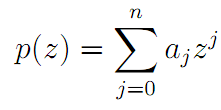be an algebraic polynomial of degree ≤n, and let ∥p∥= max {|p(z)|:|z| = 1}. We study the asymptotic behavior of the best possible constant φn,k (R), for k = 0 and k=1, in the inequality ∥p'(Rz)∥ + φn,k (R) |ak| ≤ nRn-1p∥, R → ∞.

1: Paper Source PDF document

Paper's Title:

The Best Upper Bound for Jensen's Inequality

Author(s):

Vasile Cirtoaje

Department of Automatic Control and Computers
University of Ploiesti
Romania.

Abstract:

In this paper we give the best upper bound for the weighted Jensen's discrete inequality applied to a convex function f defined on a closed interval I in the case when the bound depends on f, I and weights. In addition, we give a simpler expression of the upper bound, which is better than existing similar one.

1: Paper Source PDF document

Paper's Title:

Refinements of the Trace Inequality of Belmega, Lasaulce and Debbah

Author(s):

Shigeru Furuichi and Minghua Lin

Department of Computer Science and System Analysis,
College of Humanities and Sciences, Nihon University,
3-25-40, Sakurajyousui, Setagaya-ku, Tokyo, 156-8550, Japan.

Department of Mathematics and Statistics,

furuichi@chs.nihon-u.ac.jp, lin243@uregina.ca.

Abstract:

In this short paper, we show a certain matrix trace inequality and then give a refinement of the trace inequality proven by Belmega, Lasaulce and Debbah. In addition, we give an another improvement of their trace inequality.

1: Paper Source PDF document

Paper's Title:

Generalizing Polyhedra to Infinite Dimension

Author(s):

Paolo d'Alessandro

Department of Mathematics, Third University of Rome,
Lgo S.L. Murialdo 1, 00146 Rome, Italy.

dalex@mat.uniroma3.it.

URL: http://www.mat.uniroma3.it/users/dalex/dalex.html.

Abstract:

This paper generalizes polyhedra to infinite dimensional Hilbert spaces as countable intersections of closed semispaces. Highlights are the structure theory that shows that a polyhedron is the sum of compact set (in a suitable topology) plus a closed pointed cone plus a closed subspace, giving the internal representation of polyhedra. In the final part the dual range space technique is extended to the solution of infinite dimensional LP problems.

1: Paper Source PDF document

Paper's Title:

On an Elliptic Over-Determined Problem in Dimension Two

Author(s):

Lakhdar Ragoub
Department of Mathematics and Information of Tiyadhechnology
AL Yamamah University
P.O. Box 45 180, Riyadh 11 512
Saudi Arabia.

lragoub@yu.edu.sa

Abstract:

We extend the method of Weinberger for a non-linear over-determined elliptic problem
in R2. We prove that the domain in consideration is a ball. The tool of this investigation are maximum principles and P-functions.

1: Paper Source PDF document

Paper's Title:

Some Inequalities Concerning Derivative and Maximum Modulus of Polynomials

Author(s):

N. K. Govil, A. Liman and W. M. Shah

Department of Mathematics & Statistics,
Auburn University, Auburn,
Alabama 36849-5310,
U.S.A

Department of Mathematics,
National Institute of Technology,
Srinagar, Kashmir,
India - 190006

Department of Mathematics,
Kashmir University,
Srinagar, Kashmir,
India - 190006

Abstract:

In this paper, we prove some compact generalizations of some well-known Bernstein type inequalities concerning the maximum modulus of a polynomial and its derivative in terms of maximum modulus of a polynomial on the unit circle. Besides, an inequality for self-inversive polynomials has also been obtained, which in particular gives some known inequalities for this class of polynomials. All the inequalities obtained are sharp.

1: Paper Source PDF document

Paper's Title:

On the Inequality with Power-Exponential Function

Author(s):

Seiichi Manyama

Osaka University

Japan

manchanr4@gmail.com

Abstract:

In this paper, we prove the inequality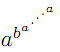<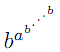for 0<a<b. Other related conjectures are also presented.

1: Paper Source PDF document

Paper's Title:

A-Normal Operators In Semi Hilbertian Spaces

Author(s):

Department of Mathematics,
College of Education for Girls in Sarat Ebeidah 61914, Abha,
King Khalid University
Saudi Arabia

Abstract:

In this paper we study some properties and inequalities of A-normal operators in semi-Hilbertian spaces by employing some known results for vectors in inner product spaces. We generalize also most of the inequalities of (α,β)-normal operators discussed in Hilbert spaces .

1: Paper Source PDF document

Paper's Title:

On Opial's Inequality for Functions of n-Independent Variables

Author(s):

S. A. A. El-Marouf and S. A. AL-Oufi

Department of Mathematics,
Faculty of Science,
Minoufiya University,
Shebin El-Koom,
Egypt

Department of Mathematics,
Faculty of Science, Taibah University,
Kingdom of Saudia Arabia

Abstract:

In this paper, we introduce Opial inequalities for functions of n-independent variables. Also, we discuss some different forms of Opial inequality containing functions of n independent variables and their partial derivatives with respect to independent variables.

1: Paper Source PDF document

Paper's Title:

Finite and Infinite Order Solutions of a Class of Higher Order Linear Differential Equations

Author(s):

Department of Mathematics,
Laboratory of Pure and Applied Mathematics,
University of Mostaganem, B. P 227 Mostaganem,
ALGERIA

Abstract:

In this paper, we investigate the growth of solutions of higher order linear differential equations where most of the coefficients have the same order and type with each other.

1: Paper Source PDF document

Paper's Title:

Equivalence of the Nonsmooth Nonlinear Complementarity Problems to Unconstrained Minimization

Author(s):

M. A. Tawhid and J. L. Goffin

Department of Mathematics and Statistics, School of Advanced Technologies and Mathematics,
Thompson Rivers University,
900 McGill Road, PO Box 3010, Kamloops, BC V2C 5N3
Alexandria University and Egypt Japan University of Science and Technology,
Alexandria-Egypt
mtawhid@tru.ca

Faculty of Management, McGill University,
1001 Sherbrooke Street West, Montreal, Quebec, H3A 1G5
Jean-Louis.Goffin@McGill.ca

Abstract:

This paper deals with nonsmooth nonlinear complementarity problem, where the underlying functions are nonsmooth which admit the H-differentiability but not necessarily locally Lipschitzian or directionally differentiable. We consider a reformulation of the nonlinear complementarity problem as an unconstrained minimization problem. We describe H-differentials of the associated penalized Fischer-Burmeister and Kanzow and Kleinmichel merit functions. We show how, under appropriate P0, semimonotone (E0), P, positive definite, and strictly semimonotone (E) -conditions on an H-differential of f, finding local/global minimum of a merit function (or a stationary point' of a merit function) leads to a solution of the given nonlinear complementarity problem. Our results not only give new results but also unify/extend various similar results proved for C1.

1: Paper Source PDF document

Paper's Title:

Fejér-type Inequalities

Author(s):

Nicuşor Minculete and Flavia-Corina Mitroi

"Dimitrie Cantemir" University,
107 Bisericii Române Street, Braşov, 500068,
România
minculeten@yahoo.com

University of Craiova, Department of Mathematics,
Street A. I. Cuza 13, Craiova, RO-200585,
Romania
fcmitroi@yahoo.com

Abstract:

The aim of this paper is to present some new Fejér-type results for convex functions. Improvements of Young's inequality (the arithmetic-geometric mean inequality) and other applications to special means are pointed as well.

1: Paper Source PDF document

Paper's Title:

Para-chaotic Tuples of Operators

Author(s):

Department of Mathematics,
Payame Noor University,
P.O. Box 19395-3697, Tehran,
Iran
b_yousefi@pnu.ac.ir

Abstract:

In this paper, we introduce para-chaotic tuples of operators and we give some relations between para-chaoticity and Hypercyclicity Criterion for a tuple of operators.

1: Paper Source PDF document

Paper's Title:

On the Boundedness of Hardy's Averaging Operators

Author(s):

Dah-Chin Luor

Department of Applied Mathematics,
I-Shou University, Dashu District,
Kaohsiung City 84001,
Taiwan, R.O.C.
dclour@isu.edu.tw

Abstract:

In this paper we establish scales of sufficient conditions for the boundedness of Hardy's averaging operators on weighted Lebesgue spaces. The estimations of the operator norms are also obtained. Included in particular are the Erdélyi-Kober operators.

1: Paper Source PDF document

Paper's Title:

On Generalized Triangle Inequality in p-Freéchet Spaces, 0<p<1

Author(s):

M. A. Latif

Department of Mathematics,
University of Hail, Hail,
Saudi Arabia
m_amer_latif@hotmail.com

Abstract:

In this paper generalized triangle inequality and its reverse in a p-Fréchet space where, 0<p<1 are obtained.

1: Paper Source PDF document

Paper's Title:

A Note on the Ulam stability of Reciprocal Difference and Adjoint Functional Equations

Author(s):

K. Ravi, J. M. Rassias, M. E. Gordji, and B. V. Senthil Kumar

Department of Mathematics,
Sacred Heart College, Tirupattur - 635601,
India
shckavi@yahoo.co.in

Pedagogical Department E. E.,
Section of Mathematics and Informatics,
National and Capodistrian University of Athens,
Athens, Attikis 15342,
GREECE
jrassias@primedu.uoa.gr

Department of Mathematics, Semnan University,
P.O. Box 35195-363, Semnan,
Iran

Department of Mathematics,
C.Abdul Hakeem College of Engineering and
Technology, Melvisharam - 632 509,
India
bvssree@yahoo.co.in

Abstract:

This note is an erratum to previous work published as Volume 8, Issue 1, Paper 13, 2011 of The Australian Journal of Mathematical Analysis and Applications.

1: Paper Source PDF document

Paper's Title:

Some Distortion and Other Properties Associated with a Family of the n-Fold Symmetric Koebe Type Functions

Author(s):

H. M. Srivastava, N. Tuneski and E. Georgieva-Celakoska

Department of Mathematics and Statistics, University of Victoria,
Victoria, British Columbia V8W 3R4,

harimsri@math.uvic.ca

Faculty of Mechanical Engineering, St.
Cyril and Methodius University,
Karpo'v s II b.b., MK-1000 Skopje,
Republic of Macedonia

Abstract:

In a recent work by Kamali and Srivastava , a certain family of the n-fold symmetric Koebe type functions was introduced and studied systematically. In an earlier investigation, Eguchi and Owa  had considered its special case when n=1 (see also ). Here, in our present sequel to these earlier works, this general family of the n-fold symmetric Koebe type functions is studied further and several distortion theorems and such other properties as the radii of spirallikeness, the radii of starlikeness and the radii of convexity, which are associated with this family of the n-fold symmetric Koebe type functions, are obtained. We also provide certain criteria that embed this family of the n-fold symmetric Koebe type functions in a function class Gλ which was introduced and studied earlier by Silverman .

1: Paper Source PDF document

Paper's Title:

Further Bounds for Two Mappings Related to the Hermite-Hadamard Inequality

Author(s):

S. S. Dragomir1,2 and I. Gomm1

1Mathematics, School of Engineering & Science,
Victoria University,
PO Box 14428, Melbourne City, MC 8001,
Australia.

2School of Computational & Applied Mathematics,
University of the Witwatersrand,
Private Bag 3, Johannesburg 2050,
South Africa.

sever.dragomir@vu.edu.au
ian.gomm@vu.edu.au
URL: http://rgmia.org/dragomir

Abstract:

Some new results concerning two mappings associated to the celebrated Hermite-Hadamard integral inequality for twice differentiable functions with applications for special means are given.

1: Paper Source PDF document

Paper's Title:

Expected Utility with Subjective Events

Author(s):

Jacob Gyntelberg and Frank Hansen

Bank for International Settlements,
Basel,
Switzerland

jacob.gyntelberg@bis.org

Tohoku University, Institute for International Education,
Sendai,
Japan

frank.hansen@m.tohoku.ac.jp

Abstract:

We provide a new theory of expected utility with subjective events modeled by a lattice of projections. This approach allows us to capture the notion of a small world'' as a context dependent or local state space embedded into a subjective set of events, the grand world''. For each situation the decision makers' subjective `small world'' reflects the events perceived to be relevant for the act under consideration. The subjective set of events need not be representable by a classical state space. Maintaining preference axioms similar in spirit to the classical axioms, we obtain an expected utility representation which is consistent across local state spaces and separates subjective probability and utility. An added benefit is that this alternative expected utility representation allows for an intuitive distinction between risk and uncertainty.

1: Paper Source PDF document

Paper's Title:

Some Functional Inequalities for the Geometric Operator Mean

Author(s):

Mustapha Raissouli

Taibah University, Faculty of Sciences, Department of Mathematics,
Al Madinah Al Munawwarah, P.O.Box 30097,
Kingdom of Saudi Arabia.

raissouli_10@hotmail.com

Abstract:

In this paper, we give some new inequalities of functional type for the power geometric operator mean involving several arguments.

1: Paper Source PDF document

Paper's Title:

New Inequalities of Mill's Ratio and Application to The Inverse Q-function Approximation

Author(s):

Pingyi Fan

Department of Electronic Engineering,
Tsinghua University, Beijing,
China

fpy@tsinghua.edu.cn

Abstract:

In this paper, we investigate the Mill's ratio estimation problem and get two new inequalities. Compared to the well known results obtained by Gordon, they becomes tighter. Furthermore, we also discuss the inverse Q-function approximation problem and present some useful results on the inverse solution. Numerical results confirm the validness of our theoretical analysis. In addition, we also present a conjecture on the bounds of inverse solution on Q-function.

1: Paper Source PDF document

Paper's Title:

Numerical Solution of A System of Singularly Perturbed Convection-Diffusion Boundary-Value Problems Using Mesh Equidistribution Technique

Author(s):

Pratibhamoy Das and Srinivasan Natesan

Department of Mathematics,
Indian Institute of Technology Guwahati,
Guwahati - 781 039,
India.

pratibhamoy@gmail.com
natesan@iitg.ernet.in
URL: http://www.iitg.ernet.in/natesan/

Abstract:

In this article, we consider a system of singularly perturbed weakly coupled convection-diffusion equations having diffusion parameters of different magnitudes. These small parameters give rise to boundary layers. An upwind finite difference scheme on adaptively generated mesh is used to obtain a suitable monitor function that gives first-order convergence which is robust with respect to the diffusion parameters. We present the results of numerical experiments for linear and semilinear system of differential equations to support the effectiveness of our preferred monitor function obtained from theoretical analysis.

1: Paper Source PDF document

Paper's Title:

An Improvement of the Hermite-Hadamard Inequality for Functions Convex on the Coordinates

Author(s):

Milica Klaričić Bakula

Faculty of Science,
University of Split,
Teslina 12, 21000 Split.
Croatia

E-mail: milica@pmfst.hr

Abstract:

An improvement of the Hermite-Hadamard inequality for functions convex on the coordinates is given.

1: Paper Source PDF document

Paper's Title:

On the Sendov Conjecture for a Root Close to the Unit Circle

Author(s):

Indraneel G. Kasmalkar

Department of Mathematics,
University of California,
Berkeley, CA 94720
United States of America

E-mail: indraneelk@berkeley.edu

Abstract:

On Sendov's conjecture, T. Chijiwa quantifies the idea stated by V. Vâjâitu and A. Zaharescu (and M. J. Miller independently), namely that if a polynomial with all roots inside the closed unit disk has a root sufficiently close to the unit circle then there is a critical point at a distance of at most one from that root. Chijiwa provides an estimate of exponential order for the required 'closeness' of the root to the unit circle so that such a critical point may exist. In this paper, we will improve this estimate to polynomial order by making major modifications and strengthening inequalities in Chijiwa's proof.

1: Paper Source PDF document

Paper's Title:

On A Conjecture of A Logarithmically Completely Monotonic Function

Author(s):

Valmir Krasniqi, Armend Sh. Shabani

Department of Mathematics,
University of Prishtina,
Republic of Kosova

E-mail: vali.99@hotmail.com
armend_shabani@hotmail.com

Abstract:

In this short note we prove a conjecture, related to a logarithmically completely monotonic function, presented in . Then, we extend by proving a more generalized theorem. At the end we pose an open problem on a logarithmically completely monotonic function involving q-Digamma function.

1: Paper Source PDF document

Paper's Title:

A Short Proof of an Open Inequality with Power-Exponential Functions

Author(s):

Mitsuhiro Miyagi and Yusuke Nishizawa

General Education, Ube National College of Technology,
Yamaguchi 755-8555,
Japan

E-mail: miyagi@ube-k.ac.jp
yusuke@ube-k.ac.jp

Abstract:

V. Cîrtoaje conjectured that a3b + b3a + ( (a -b)/2 )4 ≤ 2 holds for all nonnegative numbers a and b with a +b =2. In this short note, we give a proof of the Cîrtoaje's conjecture with power-exponential functions.

1: Paper Source PDF document

Paper's Title:

To a Banach *-algebra in a Semipartial Dynamical System

Author(s):

Bahman Tabatabaie Shourijeh and Seyed Mostafa Zebarjad

Department of Mathematics,
College of Sciences,
Shiraz University, Shiraz 71454,
Iran.

E-mail: tabataba@math.susc.ac.ir
URL: http://research.shirazu.ac.ir/faculty/More.asp?ID=207

Abstract:

By a partial dynamical system, we mean a triple containing a C*-algebra A, a discrete group G and a partial action of G on A. There are two C*--algebras associated to a given partial dynamical system. These are nothing but the certain C*-completions of a Banach *-algebra. In constructing such a Banach *-algebra, usually, a tedious limit process is used to apply. In this paper, we prove some theorems in this context without any limit process.

1: Paper Source PDF document

Paper's Title:

Some New Nonlinear Integro-Differential Inequalities of Gronwall-Bellman-Pachpatte Type

Author(s):

A. ABDELDAIM

Department of Mathematics and Computer Sciences,
Faculty of Science,
Port Said University, Port Said,
EGYPT.

Department of Mathematics,
Faculty of Science and Humanities,
SAUDI ARABIA.

E-mail: ahassen@su.edu.sa
URL: http://faculty.ksu.edu.sa/DRABDELDAIM/Pages/Home.aspx

Abstract:

In this paper we establish some new nonlinear integro-differential inequalities of Gronwall-Bellman-Pachpatte type for function of one independent variable. The purpose of this paper is to extend certain results which proved by Pachpatte in [On some fundamental integrodifferential and integral inequalities, An. Sti. Univ. Al. I. Cuza, Iasi, Vol.23 (1977), 77-86]. The inequalities obtained here can be used in the theory of some new classes of nonlinear integro-differential equations. Some applications are also given to illustrate the usefulness of our results.

1: Paper Source PDF document

Paper's Title:

A Dynamic Contact Problem for an Electro Viscoelastic Body

Author(s):

Denche M. and Ait Kaki L.

Laboratoire Equations Differentielles,
Departement de Mathematiques,
Universite Constantine 1,
Algeria.

Ecole Normale Superieure,
Departement des Sciences Exactes et Informatique,
Plateau Mansourah, Constantine.
Algeria.

Abstract:

We consider a dynamic problem which describes a contact between a piezoelectric body and a conductive foundation. The frictionless contact is modelled with the normal compliance, the electric conditions are supposed almost perfect. We prove the existence of a unique weak solution for almost perfect electric contact.

1: Paper Source PDF document

Paper's Title:

Ap Functions and Maximal Operator

Author(s):

Chunping Xie

Department of Mathematics,
Milwaukee School of Engineering,
Milwaukee, Wisconsin 53202,
U. S. A
.

E-mail: xie@msoe.edu

URL: http://www.msoe.edu/people/chunping.xie

Abstract:

The relationship between Ap functions and Hardy-Littlewood maximal operator on Lp,λ(w), the weighted Morrey space, has been studied. Also the extropolation theorem of Lp,λ(w) has been considered.

1: Paper Source PDF document

Paper's Title:

Weak solutions of non coercive stochastic Navier-Stokes equations in R2

Author(s):

Wilhelm Stannat and Satoshi Yokoyama

Technische Universität Berlin,
Strasse des 17. Juni 136, 10623 Berlin,
Germany.

The University of Tokyo,
Komaba, Tokyo 153-8914,
Japan.

E-mail: stannat@math.tu-berlin.de

E-mail: satoshi2@ms.u-tokyo.ac.jp

Abstract:

We prove existence of weak solutions of stochastic Navier-Stokes equations in R2 which do not satisfy the coercivity condition. The equations are formally derived from the critical point of some variational problem defined on the space of volume preserving diffeomorphisms in R2. Since the domain of our equation is unbounded, it is more difficult to get tightness of approximating sequences of solutions in comparison with the case of a bounded domain. Our approach is based on uniform a priori estimates on the enstrophy of weak solutions of the stochastic 2D-Navier-Stokes equations with periodic boundary conditions, where the periodicity is growing to infinity combined with a suitable spatial cutoff-technique.

1: Paper Source PDF document

Paper's Title:

C*-valued metric projection and Moore-Penrose inverse on Hilbert C*-modules

Author(s):

M. Eshaghi Gordji, H. Fathi and S.A.R. Hosseinioun

Department of Mathematics,
Semnan University, P.O. Box 35195-363, Semnan,
Iran.
Center of Excellence in Nonlinear Analysis and Applications (CENAA),
Semnan University,
Iran.

Department of Mathematics,
Shahid Beheshti University, Tehran,
Iran.
E-mail: Hedayat.fathi@yahoo.com

Department of Mathematical Sciences,
University of Arkansas, Fayetteville, Arkansas 72701,
USA.
E-mail: shossein@uark.net

Abstract:

Let t be a regular operator between Hilbert C*-modules and t be its Moore-Penrose inverse. We give some characterizations for t based on C*-valued metric projection. Moore-Penrose inverse of bounded operators and elements of a C*-algebra is studied as a special case.

1: Paper Source PDF document

Paper's Title:

Some New Generalizations of Jensen's Inequality with Related Results and Applications

Author(s):

Steven G. From

Department of Mathematics

E-mail: sfrom@unomaha.edu

Abstract:

In this paper, some new generalizations of Jensen's inequality are presented. In particular, upper and lower bounds for the Jensen gap are given and compared analytically and numerically to previously published bounds for both the discrete and continuous Jensen's inequality cases. The new bounds compare favorably to previously proposed bounds. A new method based on a series of locally linear interpolations is given and is the basis for most of the bounds given in this paper. The wide applicability of this method will be demonstrated. As by-products of this method, we shall obtain some new Hermite-Hadamard inequalities for functions which are 3-convex or 3-concave. The new method works to obtain bounds for the Jensen gap for non-convex functions as well, provided one or two derivatives of the nonlinear function are continuous. The mean residual life function of applied probability and reliability theory plays a prominent role in construction of bounds for the Jensen gap. We also present an exact integral representation for the Jensen gap in the continuous case. We briefly discuss some inequalities for other types of convexity, such as convexity in the geometric mean, and briefly discuss applications to reliability theory.

1: Paper Source PDF document

Paper's Title:

Properties of q-gamma and q-beta functions derived from the q-Gauss-Pólya inequalities

Author(s):

Sanja Varošanec

Department of Mathematics,
University of Zagreb,
Zagreb, Croatia
E-mail: varosans@math.hr

Abstract:

We consider log-convexity and other properties of several functions related to q-gamma and q-beta functions. These properties are consequences of the general inequality, so-called q-analogue of the Gauss-Pólya inequality. Various inequalities involving these special functions are also given.

1: Paper Source PDF document

Paper's Title:

Inequalities for the Area Balance of Functions of Bounded Variation

Author(s):

Sever S. Dragomir1,2

1Mathematics, School of Engineering & Science
Victoria University, PO Box 14428
Melbourne City, MC 8001,
Australia
E-mail: sever.dragomir@vu.edu.au

2DST-NRF Centre of Excellence in the Mathematical and Statistical Sciences,
School of Computer Science & Applied Mathematics,
University of the Witwatersrand,
Private Bag 3, Johannesburg 2050,
South Africa
URL: http://rgmia.org/dragomir

Abstract:

We introduce the area balance function associated to a Lebesgue integrable function f:[a,b] C by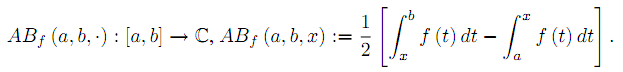Several sharp bounds for functions of bounded variation are provided. Applications for Lipschitzian and convex functions are also given.

1: Paper Source PDF document

Paper's Title:

On operators for which T2≥-T*2

Author(s):

1Department of Mathematics,
University of El Oued 39000,
Algeria
E-mail: guesbamessaoud2@gmail.com

2Department of Mathematics,
University of Msila 28000,
Algeria

Abstract:

In this paper we introduce the new class of operators for which T2 -T*2 acting on a complex Hilbert space H. We give some basic properties of these operators. we study the relation between the class and some other well known classes of operators acting on H.

1: Paper Source PDF document

Paper's Title:

Some interesting properties of finite continuous Cesŕro operators

Author(s):

Abdelouahab Mansour and Abderrazak Hechifa

Operator theory laboratory (LABTHOP),
Eloued University,
Algeria.
E-mail: amansour@math.univ-lyon1.fr

Mathematics Department,
Faculty of Science,
Algeria.
E-mail: abderrazak02@gmail.com

Abstract:

A complex scalar λ is called an extended eigenvalue of a bounded linear operator T on a complex Banach space if there is a nonzero operator X such that TX = λ XT, the operator X is called extended eigenoperator of T corresponding to the extended eigenvalue λ.

In this paper we prove some properties of extended eigenvalue and extended eigenoperator for C1 on Lp([0,1]), where C1 is the Cesŕro operator defined on the complex Banach spaces Lp([0 , 1])  for 1<p<∞ by the expression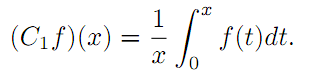1: Paper Source PDF document

Paper's Title:

Some New Inequalities of Hermite-Hadamard and Fejér Type for Certain Functions with Higher Convexity

Author(s):

Steven G. From

Department of Mathematics,
U.S.A.
E-mail: sfrom@unomaha.edu

Abstract:

In this paper, we present some new inequalities of Hermite-Hadamard or Fejér type for certain functions satisfying some higher convexity conditions on one or more derivatives.
An open problem is given also.
Some applications to the logarithmic mean are given.

1: Paper Source PDF document

Paper's Title:

Wavelet Frames in Higher Dimensional Sobolev Spaces

Author(s):

Raj Kumar, Manish Chauhan, and Reena

Department of Mathematics,
Kirori Mal College, University of Delhi,
New Delhi-110007,
India.
E-mail: rajkmc@gmail.com

Department of Mathematics,
University of Delhi,
New Delhi-110007,
India
E-mail: manish17102021@gmail.com

Department of Mathematics,
Hans Raj College, University of Delhi,
New Delhi-110007,
India
E-mail: reena.bhagwat29@gmail.com

Abstract:

In this paper, we present sufficient condition for the sequence of vectors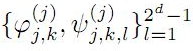to be a frame for Hs(Rd) are derived. Necessary and sufficient conditions for the sequence of vectorsto be tight wavelet frames in Hs(Rd) are obtained. Further, as an application an example of tight wavelet frames for Hs(R2) as bivariate box spline over 3-direction are given.

1: Paper Source PDF document

Paper's Title:

Euler Series Solutions for Linear Integral Equations

Author(s):

Department of Mathematics,
University of Msila 28000,
ALGERIA.
E-mail: dilmiistapha@yahoo.fr

Abstract:

In this work, we seek the approximate solution of linear integral equations by truncation Euler series approximation. After substituting the Euler expansions for the given functions of the equation and the unknown one, the equation reduces to a linear system, the solution of this latter gives the Euler coefficients and thereafter the solution of the equation. The convergence and the error analysis of this method are discussed. Finally, we compare our numerical results by others.

1: Paper Source PDF document

Paper's Title:

MSplit Equality for Monotone Inclusion Problem and Fixed Point Problem in Real Banach Spaces

Author(s):

1,2Christian Chibueze Okeke, 3Abdumalik Usman Bello, 1Chinedu Izuchukwu, and 1Oluwatosin Temitope Mewomo

1School of Mathematics,
Statistics and Computer Science,
University of KwaZulu-Natal, Durban,
South Africa.
E-mail: okekec@ukzn.ac.za
E-mail: izuchukwuc@ukzn.ac.za
E-mail: mewomoo@ukzn.ac.za

2DST-NRF Center of Excellence in Mathematical and Statistical Sciences (CoE-Mass)
Johannesburg,
South Africa.

3Federal University,
Dutsin-Ma, Katsina State,
Nigeria.
E-mail: uabdulmalik@fudutsinma.edu.ng

Abstract:

In this paper a new iterative algorithm for approximating a common solution of split equality monotone inclusion problem and split equality fixed point problem is introduced. Using our algorithm, we state and prove a strong convergence theorem for approximating an element in the intersection of the set of solutions of a split equality monotone inclusion problem and the set of solutions of a split equality fixed point problem for right Bregman strongly nonexpansive mappings in the setting of p-uniformly convex Banach spaces which are also uniformly smooth. We also give some applications.

1: Paper Source PDF document

Paper's Title:

Inequalities for Discrete F-Divergence Measures: A Survey of Recent Results

Author(s):

Sever S. Dragomir1,2

1Mathematics, School of Engineering & Science
Victoria University, PO Box 14428
Melbourne City, MC 8001,
Australia
E-mail: sever.dragomir@vu.edu.au

2DST-NRF Centre of Excellence in the Mathematical and Statistical Sciences,
School of Computer Science & Applied Mathematics,
University of the Witwatersrand,
Private Bag 3, Johannesburg 2050,
South Africa
URL: http://rgmia.org/dragomir

Abstract:

In this paper we survey some recent results obtained by the author in providing various bounds for the celebrated f-divergence measure for various classes of functions f. Several techniques including inequalities of Jensen and Slater types for convex functions are employed. Bounds in terms of Kullback-Leibler Distance, Hellinger Discrimination and Varation distance are provided. Approximations of the f-divergence measure by the use of the celebrated Ostrowski and Trapezoid inequalities are obtained. More accurate approximation formulae that make use of Taylor's expansion with integral remainder are also surveyed. A comprehensive list of recent papers by several authors related this important concept in information theory is also included as an appendix to the main text.

1: Paper Source PDF document

Paper's Title:

Relation Between The Set Of Non-decreasing Functions And The Set Of Convex Functions

Author(s):

Qefsere Doko Gjonbalaj and Luigj Gjoka

Department of Mathematics, Faculty of Electrical and Computer Engineering,
University of Prishtina "Hasan Prishtina",
Prishtine 10000,
Kosova

E-mail: qefsere.gjonbalaj@uni-pr.edu

Department of Engineering Mathematics,
Polytechnic University of Tirana, Tirana,
Albania.

E-mail: luigjgjoka@ymail.com

Abstract:

In this article we address the problem of integral presentation of a convex function. Let I be an interval in R. Here, using the Riemann or Lebesgue’s integration theory, we find the necessary and sufficient condition for a function f: I R to be convex in I.

1: Paper Source PDF document

Paper's Title:

A Comparison Between Two Different Stochastic Epidemic Models with Respect to the Entropy

Author(s):

Department of Mathematics,
University of Sussex,
Brighton BN1 9QH,
UK.
E-mail: f.fatehi@sussex.ac.uk
URL: http://www.sussex.ac.uk/profiles/361251

Department of Pure Mathematics, Faculty of Mathematics and Computer,
Shahid Bahonar University of Kerman,
Kerman 76169-14111,
Iran.

Abstract:

In this paper at first a brief history of mathematical models is presented with the aim to clarify the reliability of stochastic models over deterministic models. Next, the necessary background about random variables and stochastic processes, especially Markov chains and the entropy are introduced. After that, entropy of SIR stochastic models is computed and it is proven that an epidemic will disappear after a long time. Entropy of a stochastic mathematical model determines the average uncertainty about the outcome of that random experiment. At the end, we introduce a chain binomial epidemic model and compute its entropy, which is then compared with the DTMC SIR epidemic model to show which one is nearer to reality.

1: Paper Source PDF document

Paper's Title:

Existence of Optimal Parameters for Damped Sine-Gordon Equation with Variable Diffusion Coefficient and Neumann Boundary Conditions

Author(s):

N. Thapa

Department of Mathematical Sciences,
Cameron University,
2800 West Gore Blvd,
73505 Lawton, Oklahoma,
USA.
E-mail: nthapa@cameron.edu
URL: http://www.cameron.edu/~nthapa/

Abstract:

The parameter identification problem for sine-Gordon equation is of a major interests among mathematicians and scientists.\ In this work we the consider sine-Gordon equation with variable diffusion coefficient and Neumann boundary data. We show the existence and uniqueness of weak solution for sine-Gordon equation. Then we show that the weak solution continuously depends on parameters. Finally we show the existence of optimal set of parameters.

1: Paper Source PDF document

Paper's Title:

A kind of Function Series and Its Applications

Author(s):

Yang Tianze

Mechanical Engineering,
Shandong University, Xinglongshan Campus,
Jinan, Shandong,
China.

E-mail: qdyangtianze@163.com

Abstract:

A kind of new function series is obtained in this paper. Their theorems and proofs are shown, and some applications are given. We give the expansion form of general integral and the series expansion form of function and the general expansion form of derivative. Using them in the mathematics,we get some unexpected result.

1: Paper Source PDF document

Paper's Title:

Some Inequalities of the Hermite-Hadamard Type for k-Fractional Conformable Integrals

Author(s):

C.-J. Huang, G. Rahman, K. S. Nisar, A. Ghaffar and F. Qi

Department of Mathematics, Ganzhou Teachers College,
Ganzhou 341000, Jiangxi,
China.
E-mail: hcj73jx@126.com , huangcj1973@qq.com

Department of Mathematics, Shaheed Benazir Bhutto University,
Sheringal, Upper Dir, Khyber Pakhtoonkhwa,
Pakistan.
E-mail: gauhar55uom@gmail.com

Department of Mathematics, College of Arts and Science at Wadi Aldawaser, 11991,
Prince Sattam Bin Abdulaziz University, Riyadh Region,
Kingdom of Saudi Arabia.
E-mail: n.sooppy@psau.edu.sa, ksnisar1@gmail.com

Department of Mathematical Science, Balochistan University of Information Technology,
Engineering and Management Sciences, Quetta,
Pakistan.
E-mail: abdulghaffar.jaffar@gmail.com

School of Mathematical Sciences, Tianjin Polytechnic University,
Tianjin 300387,
China; Institute of Mathematics,
Henan Polytechnic University, Jiaozuo 454010, Henan,
China.
E-mail: qifeng618@gmail.com, qifeng618@qq.com

Abstract:

In the paper, the authors deal with generalized k-fractional conformable integrals, establish some inequalities of the Hermite-Hadamard type for generalized k-fractional conformable integrals for convex functions, and generalize known inequalities of the Hermite-Hadamard type for conformable fractional integrals.

1: Paper Source PDF document

Paper's Title:

A Generalization of Ostrowski's Inequality for Functions of Bounded Variation via a Parameter

Author(s):

Seth Kermausuor

Department of Mathematics and Computer Science,
Alabama State University,
Montgomery, AL 36101,
USA.
E-mail: skermausour@alasu.edu

Abstract:

In this paper, we provide a generalization of the Ostrowski's inequality for functions of bounded variation for k points via a parameter λ∈[0,1]. As a by product, we consider some particular cases to obtained some interesting inequalities in these directions. Our results generalizes some of the results by Dragomir in [S. S. DRAGOMIR, The Ostrowski inequality for mappings of bounded variation, Bull. Austral. Math. Soc., 60 (1999), pp. 495--508.]

1: Paper Source PDF document

Paper's Title:

Dynamical Analysis of HIV/AIDS Epidemic Model with Two Latent Stages, Vertical Transmission and Treatment

Author(s):

Nur Shofianah, Isnani Darti, Syaiful Anam

Mathematics Department,Faculty of Mathematics and Natural Sciences.
University of Brawijaya,
Jl. Veteran, Malang 65145,
Indonesia.
E-mail: nur_shofianah@ub.ac.id, isnanidarti@ub.ac.id, syaiful@ub.ac.id

Abstract:

We discuss about dynamical analysis of HIV/AIDS epidemic model with two latent stages, vertical transmission and treatment. In this model, the spreading of HIV occurs through both horizontal and vertical transmission. There is also treatment for individual who has been HIV infected. The latent stage is divided into slow and fast latent stage based on the immune condition which varies for each individual. Dynamical analysis result shows that the model has two equilibrium points: the disease-free equilibrium point and the endemic equilibrium point. The existence and global stability of equilibrium points depend on the basic reproduction number R0. When R0 <1, only the disease-free equilibrium point exists. If R0 >1, there are two equilibrium points, which are the disease-free equilibrium point and the endemic equilibrium point. Based on the result of stability analysis, the disease-free equilibrium point is globally asymptotically stable if R0 <1, while if R0 > 1 and p=q, the endemic equilibrium point will be globally asymptotically stable. In the end, we show some numerical simulations to support the analytical result.

1: Paper Source PDF document

Paper's Title:

A Low Order Least-Squares Nonconforming Finite Element Method for Steady Magnetohydrodynamic Equations

Author(s):

Z. Yu, D. Shi and H. Zhu

College of Science,
Zhongyuan University of Technology,
Zhengzhou 450007,
China.
E-mail: 5772@zut.edu.cn

School of Mathematics and Statistics,
Zhengzhou University,
Zhengzhou 450001,
China.
E-mail: shi_dy@126.com

Mathematics Department,
University of Southern Mississippi,
Hattiesburg MS, 39406,
U.S.A
E-mail: huiqing.zhu@usm.edu

Abstract:

A low order least-squares nonconforming finite element (NFE) method is proposed for magnetohydrodynamic equations with EQ1rot element and zero-order Raviart-Thomas element. Based on the above element's typical interpolations properties, the existence and uniqueness of the approximate solutions are proved and the optimal order error estimates for the corresponding variables are derived.

1: Paper Source PDF document

Paper's Title:

Action of Differential Operators On Chirpsconstruct On L

Author(s):

Taoufik El Bouayachi and Naji Yebari

Laboratoire de Mathematiques et applications,
Faculty of sciences and techniques, Tangier,
Morocco.
E-mail: figo407@gmail.com, yebarinaji@gmail.com

Abstract:

We will study in this work the action of differential operators on L chirps and we will give a new definition of logarithmic chirp. Finally we will study the action of singular integral operators on chirps by wavelet characterization and Kernel method.

1: Paper Source PDF document

Paper's Title:

Some New Mappings Related to Weighted Mean Inequalities

Author(s):

Xiu-Fen Ma

College of Mathematical and Computer,
No.9 of Xuefu Road, Hechuan District 401520,
Chongqing City,
The People's Republic of China.
E-mail: maxiufen86@163.com

Abstract:

In this paper, we define four mappings related to weighted mean inequalities, investigate their properties, and obtain some new refinements of weighted mean inequalities.

1: Paper Source PDF document

Paper's Title:

Some fixed point results in partial S-metric spaces

Author(s):

M. M. Rezaee, S. Sedghi, A. Mukheimer, K. Abodayeh, and Z. D. Mitrovic

Department of Mathematics, Qaemshahr Branch,
Iran.

Department of Mathematics, Qaemshahr Branch,
Iran.
E-mail: sedghi.gh@qaemiau.ac.ir

Department of Mathematics and General Sciences,
KSA.
E-mail: mukheimer@psu.edu.sa

Department of Mathematics and General Sciences,
KSA.
E-mail: kamal@psu.edu.sa

Nonlinear Analysis Research Group,
Faculty of Mathematics and Statistics,
Ton Duc Thang University, Ho Chi Minh City,
Vietnam.
E-mail: zoran.mitrovic@tdtu.edu.vn

Abstract:

We introduce in this article a new class of generalized metric spaces, called partial S-metric spaces. In addition, we also give some interesting results on fixed points in the partial S-metric spaces and some applications.

1: Paper Source PDF document

Paper's Title:

Inequalities for Functions of Selfadjoint Operators on Hilbert Spaces:
a Survey of Recent Results

Author(s):

Sever S. Dragomir1,2

1Mathematics, College of Engineering & Science
Victoria University, PO Box 14428
Melbourne City, MC 8001,
Australia
E-mail: sever.dragomir@vu.edu.au

2DST-NRF Centre of Excellence in the Mathematical and Statistical Sciences,
School of Computer Science & Applied Mathematics,
University of the Witwatersrand,
Private Bag 3, Johannesburg 2050,
South Africa
URL: https://rgmia.org/dragomir

Abstract:

The main aim of this survey is to present recent results concerning inequalities for continuous functions of selfadjoint operators on complex Hilbert spaces. It is intended for use by both researchers in various fields of Linear Operator Theory and Mathematical Inequalities, domains which have grown exponentially in the last decade, as well as by postgraduate students and scientists applying inequalities in their specific areas.

1: Paper Source PDF document

Paper's Title:

Oblique Projectors from the Simpson Discrete Fourier Transformation Matrix

Author(s):

P. Singh and V. Singh

School of Mathematics, Computer Science and Statistics,
University of Kwazulu-Natal,
Private Bag X54001, Durban 4001,
South Africa.
E-mail: singhp@ukzn.ac.za, singhv@ukzn.ac.za

Abstract:

In this paper we examine the projectors of the Simpson Discrete Fourier Transform matrix of dimension two modulus four and show how they decompose the complex vector space into a direct sum of oblique eigenspaces. These projection operators are used to define a Simpson Discrete Fractional Fourier Transform (SDFRFT).

1: Paper Source PDF document

Paper's Title:

Double Difference of Composition Operator on Bloch Spaces

Author(s):

Rinchen Tundup

Department of Mathematics
University of Jammu
Jammu and Kashmir
India.

E-mail: joneytun123@gmail.com

Abstract:

In this paper we characterize the compactness of double difference of three non-compact composition operators on Bloch space induced by three holomorphic self maps on the unit disc.

1: Paper Source PDF document

Paper's Title:

Accuracy of Implicit DIMSIMs with Extrapolation

Author(s):

A. J. Kadhim, A. Gorgey, N. Arbin and N. Razali

Mathematics Department, Faculty of Science and Mathematics,
Sultan Idris Education University,
35900 Tanjong Malim, Perak,
Malaysia
E-mail: annie_gorgey@fsmt.upsi.edu.my

Unit of Fundamental Engineering Studies,
Faculty of Engineering, Built Environment,
The National University of Malaysia,
43600 Bangi,
Malaysia.

Abstract:

The main aim of this article is to present recent results concerning diagonal implicit multistage integration methods (DIMSIMs) with extrapolation in solving stiff problems. Implicit methods with extrapolation have been proven to be very useful in solving problems with stiff components. There are many articles written on extrapolation of Runge-Kutta methods however fewer articles on extrapolation were written for general linear methods. Passive extrapolation is more stable than active extrapolation as proven in many literature when solving stiff problems by the Runge-Kutta methods. This article takes the first step by investigating the performance of passive extrapolation for DIMSIMs type-2 methods. In the variable stepsize and order codes, order-2 and order-3 DIMSIMs with extrapolation are investigated for Van der Pol and HIRES problems. Comparisons are made with ode23 solver and the numerical experiments showed that implicit DIMSIMs with extrapolation has greater accuracy than the method itself without extrapolation and ode23.

1: Paper Source PDF document

Paper's Title:

The Higher Coefficients for Bazilevic Functions B1(α)

Author(s):

Department of Mathematics,
Faculty of Mathematics and Natural Sciences,,
Brawijaya University, Malang Jawa Timur 65145
Indonesia.
E-mail: marjono@ub.ac.id

Abstract:

Let f be analytic in D{z: |z|< 1} with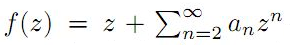, and normalized by the conditions f(0)=f'(0)-1=0. We give sharp estimates for the seventh and eighth coefficients for the class of Bazilevic functions with logarithmic growth, B1α, defined by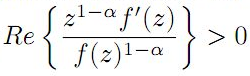for α≥0.

1: Paper Source PDF document

Paper's Title:

An Efficient Modification of Differential Transform Method for Solving Integral and Integro-differential Equations

Author(s):

S. Al-Ahmad, Ibrahim Mohammed Sulaiman*, and M. Mamat

Faculty of Informatics and Computing,
Universiti Sultan Zainal Abidin,
Terengganu, Besut Campus, 22200,
Malaysia.

Abstract:

In this paper, classes of integral and integro-differential equations are solved using a modified differential transform method. This proposed technique is based on differential transform method (DTM), Laplace transform (LT) procedure and Pad\'{e} approximants (PA). The proposed method which gives a good approximation for the true solution in a large region is referred to modified differential transform method (MDTM). An algorithm was developed to illustrate the flow of the proposed method. Some numerical problems are presented to check the applicability of the proposed scheme and the obtained results from the computations are compared with other existing methods to illustrates its efficiency. Numerical results have shown that the proposed MDTM method is promising compared to other existing methods for solving integral and integro-differential equations.

1: Paper Source PDF document

Paper's Title:

Optimization Techniques on Affine Differential Manifolds

Author(s):

Ali S Rasheed, Faik Mayah and Ahmed A H AL-Jumaili

Ministry of Higher Education and Scientific Research,
Iraq.
E-mail: ahmedhashem@gmail.com

Department of Physics, College of Sciences,
University of Wasit,
Iraq.
E-mail: faik.mayah@gmail.com

Abstract:

In addition to solid ground of Riemannian manifolds fundamentals, this article interviews some popular optimization methods on Riemannian manifolds. Several optimization problems can be better stated on manifolds rather than Euclidean space, such as interior point methods, which in turns based on self-concordant functions (logarithmic barrier functions). Optimization schemes like the steepest descent scheme, the Newton scheme, and others can be extended to Riemannian manifolds. This paper introduces some Riemannian and non-Riemannian schemes on manifolds.

1: Paper Source PDF document

Paper's Title:

Author(s):

F. U. Ogbuisi

School of Mathematics, Statistics and Computer Science,
University of KwaZulu-Natal, Durban,
South Africa.

Department of Mathematics,
University of Nigeria, Nsukka,
Nigeria.
E-mail: ferdinard.ogbuisi@unn.edu.ng fudochukwu@yahoo.com

Abstract:

In this paper, we propose and analyze a type of subgradient extragradient algorithm for the approximation of a solution of variational inequality problem which is also a common fixed point of an infinite family of relatively nonexpansive mappings in 2-uniformly convex Banach spaces which are uniformly smooth. By using the generalized projection operator, we prove a strong convergence theorem which does not require the prior knowledge of the Lipschitz constant of cost operator. We further applied our result to constrained convex minimization problem, convex feasibility problem and infinite family of equilibrium problems. Our results improve and complement related results in 2-uniformly convex and uniformly smooth Banach spaces and Hilbert spaces.

1: Paper Source PDF document

Paper's Title:

Reduced Generalized Combination Synchronization Between Two n-Dimensional Integer-Order Hyperchaotic Systems and One m-Dimensional Fractional-Order Chaotic System

Author(s):

Smail Kaouache, Mohammed Salah Abdelouahab and Rabah Bououden

Laboratory of Mathematics and their interactions,
Abdelhafid Boussouf University Center, Mila.
Algeria
E-mail: smailkaouache@gmail.com, medsalah3@yahoo.fr, rabouden@yahoo.fr

Abstract:

This paper is devoted to investigate the problem of reduced generalized combination synchronization (RGCS) between two n-dimensional integer-order hyperchaotic drive systems and one m-dimensional fractional-order chaotic response system. According to the stability theorem of fractional-order linear system, an active mode controller is proposed to accomplish this end. Moreover, the proposed synchronization scheme is applied to synchronize three different chaotic systems, which are the Danca hyperchaotic system, the modified hyperchaotic Rossler system, and the fractional-order Rabinovich-Fabrikant chaotic system. Finally, numerical results are presented to fit our theoretical analysis.

1: Paper Source PDF document

Paper's Title:

ψ(m,q)-Isometric Mappings on Metric Spaces

Author(s):

Sid Ahmed Ould Beinane, Sidi Hamidou Jah and Sid Ahmed Ould Ahmed Mahmoud

Mathematical Analysis and Applications, Mathematics Department, College of Science,
Jouf University,
Sakaka P.O.Box 2014,
Saudi Arabia.
E-mail: beinane06@gmail.com

Department of Mathematics, College of Science Qassim University,
P.O. Box 6640, Buraydah 51452,
Saudi Arabia.
E-mail: jahsiidi@yahoo.fr

Mathematical Analysis and Applications,
Mathematics Department, College of Science, Jouf University,
Sakaka P.O.Box 2014,
Saudi Arabia.
E-mail: sidahmed@ju.edu.sa, sidahmed.sidha@gmail.com

Abstract:

The concept of (m,p)-isometric operators on Banach space was extended to (m,q)-isometric mappings on general metric spaces in . This paper is devoted to define the concept of ψ(m, q)-isometric, which is the extension of A(m, p)-isometric operators on Banach spaces introduced in . Let T,ψ: (E,d) -> (E, d) be two mappings.
For some positive integer m and q (0,). T is said to be an ψ(m,q)-isometry, if for all  y,z E,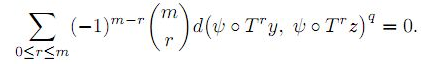1: Paper Source PDF document

Paper's Title:

Hermite-Hadamard Type Inequalities for MN-Convex Functions

Author(s):

Sever S. Dragomir1,2

1Mathematics, College of Engineering & Science
Victoria University, PO Box 14428
Melbourne City, MC 8001,
Australia
E-mail: sever.dragomir@vu.edu.au

2DST-NRF Centre of Excellence in the Mathematical and Statistical Sciences,
School of Computer Science & Applied Mathematics,
University of the Witwatersrand,
Private Bag 3, Johannesburg 2050,
South Africa
URL: https://rgmia.org/dragomir

Abstract:

The present work endeavours to briefly present some of the fundamental results connected to the Hermite-Hadamard inequality for special classes of convex functions such as AG, AH, GA, GG, GH, HA, HG and HH -convex functions in which the author have been involved during the last five years. For simplicity, we call these classes of functions such as MN-convex functions, where M and N stand for any of the Arithmetic (A), Geometric (G) or Harmonic (H) weighted means of positive real numbers. The survey is intended for use by both researchers in various fields of Approximation Theory and Mathematical Inequalities, domains which have grown exponentially in the last decade, as well as by postgraduate students and scientists applying inequalities in their specific areas.

1: Paper Source PDF document

Paper's Title:

Sweeping Surfaces with Darboux Frame in Euclidean 3-space E3

Author(s):

F. Mofarreh, R. Abdel-Baky and N. Alluhaibi

Mathematical Science Department, Faculty of Science,
Princess Nourah bint Abdulrahman University
Saudi Arabia.
E-mail: fyalmofarrah@pnu.edu.sa

Department of Mathematics, Faculty of Science,
University of Assiut,
Assiut 71516,
Egypt.
E-mail: rbaky@live.com

Department of Mathematics Science and Arts, College Rabigh Campus,
King Abdulaziz University
Jeddah,
Saudi Arabia.
E-mail: nallehaibi@kau.edu.sa

Abstract:

The curve on a regular surface has a moving frame and it is called Darboux frame. We introduce sweeping surfaces along the curve relating to the this frame and investigate their geometrical properties. Moreover, we obtain the necessary and sufficient conditions for these surfaces to be developable ruled surfaces. Finally, an example to illustrate the application of the results is introduced.

1: Paper Source PDF document

Paper's Title:

Construction of a Frame Multiresolution Analysis on Locally Compact Abelian Groups

Author(s):

R. Kumar and Satyapriya

Department of Mathematics,
Kirori Mal College,
University of Delhi,
Delhi,
India.
E-mail: rajkmc@gmail.com

Department of Mathematics,
University of Delhi,
Delhi,
India.
E-mail: kmc.satyapriya@gmail.com

Abstract:

The frame multiresolution analysis (FMRA) on locally compact Abelian groups has been studied and the results concerning classical MRA have been worked upon to obtain new results. All the necessary conditions, which need to be imposed on the scaling function φ to construct a wavelet frame via FMRA, have been summed up. This process of construction of FMRA has aptly been illustrated by sufficient examples.

1: Paper Source PDF document

Paper's Title:

Some criteria for Subspace-hypercyclicity of C0-semigroups

Author(s):

Mansooreh Moosapoor

Department of Mathematics,
Farhangian University, Tehran,
Iran.
E-mail: m.mosapour@cfu.ac.ir
mosapor110@gmail.com

Abstract:

We research subspace-hypercyclic C0-semigroups in this paper. We present various types of subspace-hypercyclicity criteria for C0-semigroups. Some of them are stronger than the criteria introduced before. Also, we state that if a C0-semigroup (Tt}t 0 satisfies in any of them, then (Tt⊕Tt}t 0 is subspace-hypercyclic.

1: Paper Source PDF document

Paper's Title:

Evaluation of a New Class of Double Integrals Involving Generalized Hypergeometric Function 4F3

Author(s):

Joohyung Kim, Insuk Kim and Harsh V. Harsh

Department of Mathematics Education,
Wonkwang University, Iksan, 570-749,
Korea.
E-mail: joohyung@wku.ac.kr

Department of Mathematics Education,
Wonkwang University, Iksan, 570-749,
Korea.
E-mail: iki@wku.ac.kr

Department of Mathematics, Amity School of Eng. and Tech.,
Amity University Rajasthan
NH-11C, Jaipur-303002, Rajasthan,
India.
E-mail: harshvardhanharsh@gmail.com

Abstract:

Very recently, Kim evaluated some double integrals involving a generalized hypergeometric function 3F2 with the help of generalization of Edwards's well-known double integral due to Kim,  et al. and generalized classical Watson's summation theorem obtained earlier by Lavoie,  et al. In this research paper we evaluate one hundred double integrals involving generalized hypergeometric function 4F3 in the form of four master formulas (25 each) viz. in the most general form for any integer. Some interesting results have also be obtained as special cases of our main findings.

1: Paper Source PDF document

Paper's Title:

Some Remarks On Quasinearly Subharmonic Functions

Author(s):

Mansour Kalantar

Universite Toulouse III-Paul Sabatier,
118 Route de Narbonne, 31062 Toulouse,
France.
E-mail: mansour.kalantar@math.univ-toulouse.fr, mankalantar12@yahoo.com

Abstract:

We prove some basic properties of quasi-nearly subharmonic functions and quasi-nearly subharmonic functions in the narrow sense.

1: Paper Source PDF document

Paper's Title:

Nonlinear System of Mixed Ordered Variational Inclusions Involving XOR Operation

Author(s):

Iqbal Ahmad, Abdullah and Syed Shakaib Irfan

Department of Mechanical Engineering,
College of Engineering, Qassim University
Buraidah 51452, Al-Qassim,
Saudi Arabia.

Zakir Husain Delhi College,
University of Delhi,
JLN Marg, New Delhi- 110 002,
India.
E-mail: abdullahdu@qec.edu.sa

Department of Mathematics,
Aligarh Muslim University, Aligarh,
India.
E-mail: shakaibirfan@gmail.com

Abstract:

In this work, we introduce and solve an NSMOVI frameworks system involving XOR operation with the help of a proposed iterative algorithm in real ordered positive Hilbert spaces. We discuss the existence of a solution of a considered system of inclusions involving XOR operation by applying the resolvent operator technique with XOR operation and also study the strong convergence of the sequences generated by the considered algorithm. Further, we give a numerical example in support of our considered problem which gives the grantee that all the proposed conditions of our main result are fulfilled.

1: Paper Source PDF document

Paper's Title:

Semivectorial Bilevel Optimization on Affine-Finsler-Metric Manifolds

Author(s):

Faik Mayah1, Ali S Rasheed2 and Naseif J. Al- Jawari3

1Department of Physics,
College of Sciences,
University of Wasit,
Iraq.
E-mail: faik.mayah@gmail.com

2Ministry of Higher Education and Scientific Research,
Iraq.
E-mail: ali.math2018@yahoo.com ahmedhashem@gmail.com

3Dept. of Mathematics,
College of Science,
Iraq.
E-mail: nsaif642014@yahoo.com

Abstract:

A Finsler manifold is a differential manifold together with a Finsler metric, in this paper we construct a new class of Finsler metric affine manifolds on bilevel semivectorial with optimization problems. The first steps for this purpose involve the study of bilevel optimization on affine manifolds. The bilevel programming problem can be viewed as a static version of the noncooperative, two-person game which was introduced in the context of unbalanced economic markets. Bilevel optimization is a special kind of optimization where one problem is embedded within another.

1: Paper Source PDF document

Paper's Title:

Coefficient Estimates Of Sakaguchi Kind Functions Using Lucas Polynomials

Author(s):

H. Priya and B. Srutha Keerthi

Department of Mathematics,
VIT Chennai Campus,
Chennai - 600 048,
India.
E-mail: priyaharikrishnan18@gmail.com

Department of Mathematics,
VIT Chennai Campus,
Chennai - 600 048,
India.
E-mail: i
sruthilaya06@yahoo.co.in

Abstract:

By means of (p,q) Lucas polynomials, we estimate coefficient bounds and Fekete-Szego inequalities for functions belonging to this class. Several corollaries and consequences of the main results are also obtained.

1: Paper Source PDF document

Paper's Title:

Hankel Functional Connected to Lemniscate of Bernoulli

Author(s):

K. Ramanuja Rao, Rajnesh Lal and Kaushal Singh

Fiji National University,
Department of Mathematics & Statistics,
P.O. Box 5529, Lautoka,
Fiji.
E-mail: ramanuja.kotti@fnu.ac.fj
rajnesh.lal@fnu.ac.fj
kaushal.singh@fnu.ac.fj

Abstract:

The aim of present paper is to derive a higher bound (HB) of 3rd order Hankel determinant for a collection of holomorphic mappings connected with exactly to the right side of the lemniscate of Bernoulli, whose polar coordinates form is r2 = 2cos2(2θ). The method carried in this paper is more refined than the method adopted by the authors (see ), who worked on this problem earlier.

1: Paper Source PDF document

Paper's Title:

A New Method with Regularization for Solving Split Variational Inequality Problems in Real Hilbert Spaces

Author(s):

Francis Akutsah1 and Ojen Kumar Narain2

1School of Mathematics,
Statistics and Computer Science,
University of KwaZulu-Natal, Durban,
South Africa.
E-mail: 216040405@stu.ukzn.ac.za, akutsah@gmail.com

2School of Mathematics,
Statistics and Computer Science,
University of KwaZulu-Natal, Durban,
South Africa.
E-mail: naraino@ukzn.ac.za

Abstract:

In this paper, we introduce a new inertial extrapolation method with regularization for approximating solutions of split variational inequality problems in the frame work of real Hilbert spaces. We prove that the proposed method converges strongly to a minimum-norm solution of the problem without using the conventional two cases approach. In addition, we present some numerical experiments to show the efficiency and applicability of the proposed method. The results obtained in this paper extend, generalize and improve several results in this direction.

1: Paper Source PDF document

Paper's Title:

Multistage Analytical Approximate Solution of Quasi-Linear Differential- Algebraic System of Index Two

Author(s):

Ibrahim M. Albak, F. A. Abdullah* and Zarita Zainuddin

School of Mathematical Sciences,
Universiti Sains Malaysia,
11800 USM, Penang,
Malaysia.
E-mail:
ibra13975@gmail.com,
farahaini@usm.my,
zarita@usm.my

Abstract:

In this paper, a new Multistage Transform Method (MSDTM) has been proposed by utilizing a well-known transformation technique, the Differential Transform Method (DTM), to solve Differential Algebraic Equations (DAEs) with index 2. The advantage of the proposed scheme is that it does not require an index reduction and extends the convergence domain of the solution. Some examples for various types of problems are carried out to show the ability of MSDTM in solving DAEs. The results obtained are in good agreement with the existing literature which demonstrates the effectiveness and efficiency of the proposed method.

1: Paper Source PDF document

Paper's Title:

A Review on Minimally Supported Frequency Wavelets

Author(s):

K Pallavi1, M C Lineesh1, A Noufal2

1Department of Mathematics,
National Institute of Technology Calicut,
Kerala 673601,
India.
E-mail:
pavikrishnakumar@gmail.com
lineesh@nitc.ac.in

2Department of Mathematics,
Cochin University of Science and Technology,
Kerala 682022,
India.
E-mail: noufal@cusat.ac.in

Abstract:

This paper provides a review on Minimally Supported Frequency (MSF) wavelets that includes the construction and characterization of MSF wavelets. The characterization of MSF wavelets induced from an MRA is discussed and the nature of the low-pass filter associated with it is explained. The concept of wavelet set and dimension function is introduced to study this class of wavelets. Along with MSF wavelets, s-elementary wavelets and unimodular wavelets are also considered due to the similarity in definitions. Examples and illustrations are provided for more clarity.

1: Paper Source PDF document

Paper's Title:

On General Class of Nonlinear Contractive Maps and their Performance Estimates

Author(s):

Olalekan Taofeek Wahab and Salaudeen Alaro Musa

Department of Mathematics and Statistics
Kwara State University, Malete
P. M. B. 1530 Ilorin,
Nigeria.
E-mail: taofeek.wahab@kwasu.edu.ng

Abstract:

This paper considers two independent general class of nonlinear contractive maps to study the existence properties of nonlinear operators with prior degenerate. The existence properties are proved in the framework of approximate fixed points with the imposition of the general class of contractive conditions in metrical convex spaces without emphasis on completeness or compactness. For computational purposes, the performance estimates and the sensitivity dependence of these conditions are obtained for the Picard operator. Practical examples are also considered to justify the validity of the conditions. The results ensure no term is lost in the operators with prior degenerate and the conditions are strictly larger class when compare with others in the literature.

1: Paper Source PDF document

Paper's Title:

Error Bounds for Numerical Integration of Functions of Lower Smoothness and Gauss-Legendre Quadrature Rule

Author(s):

Samuel A. Surulere and Abiola O. Oladeji

Tshwane University of Technology
Department of Mathematics and Statistics
175, Nelson Mandela drive, Arcadia, Pretoria,
South Africa.
E-mail: samuel.abayomi.sas@gmail.com

Abstract:

The error bounds of the rectangular, trapezoidal and Simpson's rules which are commonly used in approximating the integral of a function (f(x)) over an interval ([a,b]) were estimated. The error bounds of the second, and third generating functions of the Gauss-Legendre quadrature rules were also estimated in this paper. It was shown that for an (f(t)) whose smoothness is increasing, the accuracy of the fourth, sixth and eighth error bound of the second, and third generating functions of the Gauss-Legendre quadrature rule does not increase. It was also shown that the accuracy of the fourth error bound of the Simpson's (1/3) and (3/8) rules does not increase.

1: Paper Source PDF document

Paper's Title:

Trace Inequalities for Operators in Hilbert Spaces: a Survey of Recent Results

Author(s):

Sever S. Dragomir1,2

1Mathematics, School of Engineering & Science
Victoria University,
PO Box 14428 Melbourne City, MC 8001,
Australia
E-mail: sever.dragomir@vu.edu.au

2DST-NRF Centre of Excellence in the Mathematical and Statistical Sciences,
School of Computer Science & Applied Mathematics,
University of the Witwatersrand,
Private Bag 3, Johannesburg 2050,
South Africa
URL: https://rgmia.org/dragomir

Abstract:

In this paper we survey some recent trace inequalities for operators in Hilbert spaces that are connected to Schwarz's, Buzano's and Kato's inequalities and the reverses of Schwarz inequality known in the literature as Cassels' inequality and Shisha-Mond's inequality. Applications for some functionals that are naturally associated to some of these inequalities and for functions of operators defined by power series are given. Further, various trace inequalities for convex functions are presented including refinements of Jensen inequality and several reverses of Jensen's inequality. Hermite-Hadamard type inequalities and the trace version of Slater's inequality are given. Some Lipschitz type inequalities are also surveyed. Examples for fundamental functions such as the power, logarithmic, resolvent and exponential functions are provided as well.

1: Paper Source PDF document

Paper's Title:

Reverse Hölder and Minkowski type integral inequalities for n functions

Author(s):

Panagiotis T. Krasopoulos and Lazhar Bougoffa

Department of Informatics, KEAO,
Electronic National Social Security Fund,
12 Patision St., 10677, Athens,
Greece.
E-mail: pan_kras@yahoo.gr
pankras@teemail.gr

Department of Mathematics,
Faculty of Science, Imam Mohammad Ibn Saud Islamic University,
Saudi Arabia.
E-mail: lbbougoffa@imamu.edu.sa
bougoffa@hotmail.com

Abstract:

We present and prove new reverse Hölder and Minkowski type integral inequalities for n functions. We compare our results with other known results from the relative literature in order to test their performance. In this respect, our theorems can be viewed as generalizations of some already known integral inequalities.

1: Paper Source PDF document

Paper's Title:

Conservativeness Criteria of Girsanov Transformation for Non-Symmetric Jump-diffusion

Author(s):

Mila Kurniawaty

DDepartment of Mathematics,
Universitas Brawijaya, Malang,
Indonesia.
E-mail: mila_n12@ub.ac.id

Abstract:

We develop the condition in our previous paper [The Conservativeness of Girsanov transformed for symmetric jump-diffusion process (2018)] in the framework of nonsymmetric Markov process with jumps associated with regular Dirichlet form. We prove the conservativeness of it by relation in duality of Girsanov transformed process and recurrent criteria of Dirichlet form.

1: Paper Source PDF document

Paper's Title:

A Caratheodory's Approximate Solutions of Stochastic Differential Equations Under the Hölder Condition

Author(s):

Bo-Kyeong Kim and Young-Ho Kim

Department of Mathematics,
Changwon National University,
Changwon, Gyeongsangnam-do 51140,
Korea.
E-mail: claire9576@naver.com
yhkim@changwon.ac.kr

Abstract:

In this paper, based on the theorem of the uniqueness of the solution of the stochastic differential equation, the convergence possibility of the Caratheodory's approximate solution was studied by approximating the unique solution. To obtain this convergence theorem, we used a Hölder condition and a weakened linear growth condition. Furthermore, The auxiliary theorems for the existence and continuity of the Caratheodory's approximate solution were investigated as a prerequisite.

1: Paper Source PDF document

Paper's Title:

Corrigendum for Multistage Analytical Approximate Solution of Quasi-Linear Differential- Algebraic System of Index Two

Author(s):

Ibrahim M. Albak, F. A. Abdullah* and Zarita Zainuddin

School of Mathematical Sciences,
Universiti Sains Malaysia,
11800 USM, Penang,
Malaysia.
E-mail:
ibra13975@gmail.com,
farahaini@usm.my,
zarita@usm.my

Abstract:

1: Paper Source PDF document

Paper's Title:

A Fuzzy Soft Quotient Topology and Its Properties

Author(s):

Haripamyu, Riri Alfakhriati, Monika Rianti Helmi, Jenizon

Department of Mathematics and Data Science, Andalas University, Padang, Indonesia.
E-mail: haripamyu@sci.unand.ac.id
ririalfakhriati123@gmail.com
monikariantihelmi@sci.unand.ac.id
jenizon@gmail.com

Abstract:

This research is to construct a new topology on fuzzy soft set by using the concept of quotient topology. Then we study the concept of quotient map to define the fuzzy soft quotient map and provide some relevant properties of fuzzy soft quotient map. Furthermore, we give some examples related to fuzzy soft quotient topology and fuzzy soft quotient map to apply some properties of fuzzy soft quotient map.

1: Paper Source PDF document

Paper's Title:

Two Geometric Constants Related to Isosceles Orthogonality on Banach Space

Author(s):

Huayou Xie, Qi Liu and Yongjin Li

Department of Mathematics,
Sun Yat-sen University,
Guangzhou, 510275,
P. R. China.
E-mail: xiehy33@mail2.sysu.edu.cn

Department of Mathematics,
Sun Yat-sen University,
Guangzhou, 510275,
P. R. China.
E-mail: liuq325@mail2.sysu.edu.cn

Department of Mathematics,
Sun Yat-sen University,
Guangzhou, 510275,
P. R. China.
E-mail: stslyj@mail.sysu.edu.cn

Abstract:

In this paper, we introduce new geometric constant C(X,ai,bi,ci,2) to measure the difference between isosceles orthogonality and special Carlsson orthogonalities. At the same time, we also present the geometric constant C(X,ai,bi,ci), which is a generalization of the rectangular constant proposed by Joly. According to the inequality on isosceles orthogonality, we give the boundary characterization of these geometric constants. Then the relationship between these geometric constants and uniformly non-square property can also be discussed. Furthermore, we show that there is a close relationship between these geometric constants and some important geometric constants.

1: Paper Source PDF document

Paper's Title:

Fekete Szegö problem on the Class of Bazilevič functions B1(α) related to the Lemniscate Bernoulli

Author(s):

N. M. Asih, Marjono, Sa'adatul Fitri, Ratno Bagus Edy Wibowo

Department of Mathematics,
University of Brawijaya,
Malang 65145,
Indonesia.
Department of Mathematics,
University of Udayana,
Bali,
Indonesia.

Department of Mathematics,
University of Brawijaya,
Malang 65145,
Indonesia.
E-mail: marjono@ub.ac.id

Department of Mathematics,
University of Brawijaya,
Malang 65145,
Indonesia.

Department of Mathematics,
University of Brawijaya,
Malang 65145,
Indonesia.
E-mail: rbagus@ub.ac.id

Abstract:

We provide a sharp boundaries inequalities for Fekete Szegö problem |a3-μ a22|, the coefficients of logarithmic function log~ f(z)/z, and the coefficients of the inverse function f(f'(w)) on the Bazilevič functions B1(α) related to the Lemniscate Bernoulli on the unit disk D={z: |z| < 1}. We obtained the result by using some properties of function with positive real part relates to coefficients problems.

1: Paper Source PDF document

Paper's Title:

Bicomplex Univalent Functions

Author(s):

Mohd Arif, Amjad Ali, Rajat Singh* and Romesh Kumar

Department of Mathematics,
University of Jammu,
Jammu And Kashmir,
India.
E-mail: azizymaths@gmail.com

Department of Mathematics,
University of Jammu,
Jammu And Kashmir,
India.

Department of Mathematics,
University of Jammu,
Jammu And Kashmir,
India.
E-mail: *rajat.singh.rs634@gmail.com

Department of Mathematics,
University of Jammu,
Jammu And Kashmir,
India.
E-mail: romeshmath@gmail.com

Abstract:

In this paper we introduce bicomplex univalent functions and also discuss the properties of a specific class of univalent functions.

Search and serve lasted 0 second(s).